## A Chess Puzzle, Part VIII Conclusion: The Transfinite Super Queen and BeyondFinal Conclusion of the journey of A Mathematical Chess Puzzle:

### Journey’s End

It has been a long journey, but with this post the story of the Mathematical Chess Puzzle will be coming to an end.

When I first sat down in the waiting room at Southern Utah’s Intermountain Health Care on May 19, 2019, and stared up at the infinite array of square acoustic tiles in the ceiling, the 2020 election was still over a year away. There was no worldwide pandemic that had killed off a million people worldwide and affected a third of everybody I knew. The company I had worked at since 1995 was still my employer. The company I worked for next did not yet even exist. Our best friend in town had not yet died tragically of a stroke. Her cats who had been rendered homeless had not come to live with us. There was no Russian invasion and war with Ukraine. There was no attempted overthrow of the US government. There were no Llamas in our back yard named Dewi and Karl, clearing out weeds. My wife had not yet been convinced to run and then win the race to become Mayor of the town of Virgin. And up until today (June 12 2022), my wife had not contracted Covid. She’s doing ok.

Yet through all of this, and more, I would steal time from work, and grab some blank paper, and sketch some chess positions, look up articles, refresh my knowledge of group theory and work on each chess piece, to understand both its power and its “density” as a result of that power.

We would travel to Gigi’s siblings houses in Austin Texas, I would lie on the couch, and scribble some more diagrams, then go to Chuy’s restaurant, still thinking about the problems, hoping to remember some flash of insight about the Rook, through all the margaritas I had just drank.

This last leg of the journey was the longest. In my line of mathematics I had not heard of Transfinite Induction, and the bizarre amazing results that could be proven with it (as sensible as the Axiom of Choice may seem). It was not at all clear to me at the beginning that I would be able to solve this problem, but yet I did.

Let us then begin the end at the beginning, with the story of Numbers. This story is going to go to some strange places, but to end the story, we have to go there. So humor me …

### Numbers, Ordinal and Cardinal

In order to attack the Super Queen Theorem, we will need to make use of the properties of ordinal and cardinal numbers. Most folks understand on some level the difference between these two. Cardinal numbers simply say how many things there are, while Ordinal numbers pay much closer attention to the order – of which ones come first. In other words, it is the difference between five sheep and the fifth sheep (or the sixth sick sheikh’s sixth sheep — which I hear is ailing). So the Cardinal numbers go Zero, One, Two Three, while the Cardinal numbers go Zeroth, First, Second, Third.

Things however become much more complex (and possibly confusing) once you start dealing with infinite sets of things. For example, if you consider the set of Whole numbers $\mathbb{W} = \{0, 1, 2, …\}$, the members have the property that they are ordered, and that if m and n are distinct elements, then either m > n or n > m. Now suppose you create a new set $\mathbb{W}^*$, which is all of the elements of $\mathbb{W}$, together with a new member called $\omega$ (omega), for which it is understood that $\omega > n$ for all whole numbers $n$. You can then define addition on this new set, with numbers like $\omega + 1$, $\omega + \omega = 2\omega$ and so on. It turns out that each of these are good *ordinal* numbers and you can always define the “>” operator in a consistent way. But even with all these new ordinals, it turns out that in terms of the *size* of these new sets, they both have the same number of elements, which is $\aleph_0$. It is only when you extend the whole numbers to real numbers, that suddenly the cardinality increases, to $c$, the cardinality of the continuum, which is strictly greater than $\aleph_0$.

Note: for a good expository article on transfinite induction and its uses, see Sylvia Durian’s article, “Some Transfinite Induction Deductions“, which was part of the University of Chigago Math department’s “Research Experience for Undergraduate” (REU) program.

### Transfinite Induction

The relationship between ordinal numbers and (regular) mathematical induction is fairly clear. In order to prove some statement S(n) for all whole numbers n, you first prove S(0), and then prove that if S is true for the nth value, then it is true for the (n+1)th value. In other words, you have to prove the statements in order, and from there you can go to $\omega$.

The idea for transfinite induction is basically the same, except that you are iterating not just on finite $n$ steps, but on steps $\alpha$, where $\alpha$ may be an ordinal which is already itself infinite.

Because of the delicate nature of this transfinite arithmetic, the way in which “mathematical induction” must be extended to such numbers must be handled differently. In order to do this, we have to distinguish between different kinds of “ordinal” numbers, some finite, some infinite, and some more infinite than others such as $\omega^2, \omega^\omega$, etc. See the diagram below.

### Successor Ordinals and Limit Ordinals

So consider our extended “Whole” numbers $\mathbb{W}^*$ above where we have added a new “infinite” number $\omega$, whose property is that it is greater than all finite whole numbers. The number $\omega$ is different from the finite ones in an important way: given any positive finite ordinal $n$, it has a predecessor, $n-1$ as well as a successor $n+1$. Unlike these, the number $\omega$ has no immediate predecessor, though it does have successors $\omega +1, \omega+2$ etc. Ordinals with no predecessors are called limit ordinals. Ordinals which do have predecessors are called successor ordinals. By this definition, note also that the first whole number $0$ is a limit ordinal.

### The Principle of Transfinite Induction

In order to prove statement $S(\alpha)$ for all $\alpha$ in some infinite index space $\Lambda$, you must prove not two but three things (see wikipedia):

1. Zero Case: Prove that $S(0)$ is true
2. Successor case: Prove that for any successor ordinal $\alpha +1 \in \Lambda$, that $S(\alpha + 1)$ follows from $S(\alpha)$
3. Limit case: Prove that for any limit ordinal $\lambda$, $S(\lambda)$ follows from $S(\beta)$ for all $\beta \lt \lambda$.

It is this last step that is the new twist, which we did not have to do in the finite “rational board” case.

We will also need one other important item for our toolkit.

(Ernest) Zermelo’s Theorem: Every set $S$ can be well-ordered. That is, every element in $S$ can be assigned an index or label $\alpha$, and an ordering $\le$ such that $\{ \alpha, \le \}$ is a well ordered set.

These two theorems are well known, and we will not prove them here. The latter is a direct consequence of the Axiom of Choice.

Definition: In any subset $S \subset \mathbb{R}^2$, the queen lines $\Lambda(S)$ of $S$ are the intersection with $S$ of all lines of the form $ax + by = c$, where $(|a|,|b|)$ belongs to the set $\{ (1,0), (0,1), (1,1) \}$ and $c$ is any real number. In other words the queen lines are any horizontal, vertical, or diagonal lines in the plane.

The Transfinite Super Queen Theorem: In the open unit board $\mathbb{B}^* = \mathbb{B}_{I^*}$, where $I^* = (0,1)$, there exists a “position” (set of points) $Q \subset \mathbb{B}^*$ such that every queen line of $\mathbb{B}^*$ meets $Q$ in exactly one point.

PROOF: Let $\Lambda = \Lambda(\mathbb{B}^*)$ be the set of all queen lines $\lambda$ that intersect the unit board $\mathbb{B}^*$, and let $\{ \lambda_{\alpha} \}$ be a well-ordered labeling of that set using ordinals $\alpha$ (which we know exists by Zermelo’s Theorem). We will use transfinite induction on $\alpha$ to construct a sequence $\{ Q_\alpha \}$ of sets $Q_\alpha \subset \mathbb{B}^*$, such that for every $\alpha$, the following properties hold:

i) $Q_\alpha$ contains at most one point.

ii) $\bigcup\limits_{\beta \le \alpha} Q_{\alpha}$ does not contain any pairs of points belonging to the same queen line.

iii) $\bigcup\limits_{\beta \le \alpha} Q_{\alpha}$ contains exactly one point of $\lambda_\alpha$.

Following the principle of Transfinite Induction, we must consider three cases. The Zero Case — to get started, the Successor Case — which gets us to a step having a preceeding step, and the Limit Cases. This latter is the new one, and are those infinitely far away steps where there is no immediate preceeding step, but an infinity of previous ones, even an infinity of infinity of them.

Zero Case: We arbitrarily pick any point $q_0 \in \lambda_0$, and define $Q_0 = \{ q_0 \}$. The three properties (i)-(iii) are seen to hold trivially.

Successor Case: Suppose for successor ordinal $\alpha + 1$ the sequence $\{ Q_\beta\}_{\beta \le \alpha}$ satisfies properties (i)-(iii) for $\alpha$. We must construct (and prove) that there exists a set $Q_{\alpha +1}$ for which those properties also hold.

Consider the set $Q = \bigcup\limits_{\beta \le \alpha} Q_{\alpha}$. By (ii) we know that it does not contain any pairs of points belonging to any queen lines, including $\lambda_{\alpha +1}$. There are therefore just two cases to consider:

Case 1: If $|Q \cap \lambda_{\alpha +1}| = 1$, define $Q_{\alpha + 1} = \emptyset$. This clearly satisfies all three conditions for $\alpha + 1$.
Case 2: if the intersection is empty, define $Q_{\alpha + 1} = \{q\}$, where $q \in \lambda_{\alpha + 1}$ is chosen by the following process:

Let $Q = \bigcup\limits_{\beta \le \alpha} Q_{\alpha}$ and define $\Lambda(Q)$, to be the set of all queen lines passing through Q. Now through any point only four distinct queen lines can pass, and so the intersection of $\Lambda(Q)$ with queen line $\lambda_{\alpha + 1}$  has cardinality no more than

$$4*|Q| \lt 2^{\aleph_0} = |\lambda_{\alpha + 1}|$$

in other words, the set

$$\lambda_{\alpha + 1} \setminus \Lambda(Q) \ne \emptyset$$

Hence we can choose $q \in \lambda_{\alpha + 1}$ to be any element in this remaining set, and define $Q_{\alpha +1} = \{q\}$. We assert that this satisfies all three conditions (i)-(iii). This follows immediately by the construction itself.

This diagram below may help clarify the rather complex chain of arguments above: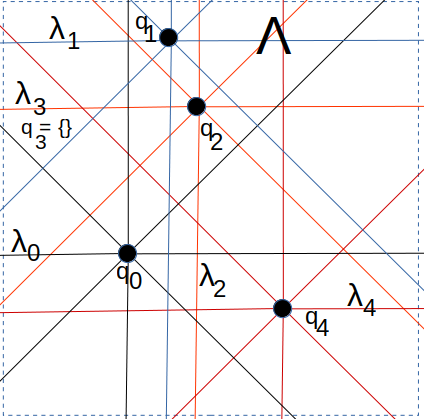The small lambdas are the successive queen lines in the well-ordered sequence. For line $\lambda_0$ we chose an arbitrary point $q_0$ on it. Inspecting $\lambda_1$ we see it does not intersect $Q = \{q_0\}$ and so we are in Case 2 and we carefully choose $q_1$ to be a point not in the intersection of $\lambda_1$ with any queen lines passing through $q_0$. For $\lambda_2$ we have the same case and again we define $q_2$ to be a point not in the intersection of $\lambda_2$ with any queen lines through $\{q_0, q_1\}$. Moving on to $\lambda_3$, we see that it actually intersects with $q_2$, meaning that we are in Case 1. In this case, we simply define $Q_3 = \{\}$, the empty set, and move on to $\lambda_4$, which is back to Case 2.

To finish this proof, however, we still have one more case, which is for limit ordinals (a case which does not occur in finite induction).

Limit Case: We now consider the case where $\alpha$ is a limit ordinal with no immediate predecessor, and where all that we know is that conditions i-iii hold for all ordinals $\beta < \alpha$. We must show that we can find a set $Q_\alpha$ which also satisfies the conditions for $\lambda_\alpha$.

Consider now the collection of nested sets $S_\gamma = \bigcup\limits_{\beta \le \gamma} Q_{\gamma}$, for $\gamma \lt \alpha$.  We know that by (ii) none of them contain any pairs of points on the same queen line. We assert that their union

$$Q = \bigcup\limits_{\gamma \lt \alpha} S_{\gamma} = \bigcup\limits_{\beta \lt \alpha} Q_{\gamma}$$

has the same property and contains no pairs of points on any queen line. We will prove this by contradiction. To see this, consider the set $T \subset \Lambda$, defined to be the set of all queen lines (if they exist) for which $Q$ does contain a pair of points as members. As a well-ordered set $T$ must have a member with the lowest index, call it $\lambda_\epsilon$. This queen line is presumed to meet $Q$ in two points, call them $p$ and $q$. As members of $Q$ which are unions of $S_\beta$, these two must be members of two specific $S_\beta$, call them $\beta_p$ and $\beta_q$. Let $\kappa$ be the larger of these two. Since the $S_\gamma$ are nested, this means that both $p$ and $q$ are in $S_\kappa$.  But $\kappa \lt \alpha$, and so we know that $S_\kappa$ satisfies condition (ii), a contradiction.

Thus we conclude that $Q$ satisfies (ii) in the sense that it contains no pairs of any queen line. Let us now define $Q_\alpha$ to satisfy (i-iii). We know that $Q$ does not contain a pair of points in $\lambda_\alpha$ and so we have once again two cases:

Case 1: If $|Q \cap \lambda_{\alpha}| = 1$, define $Q_{\alpha} = \emptyset$. This clearly satisfies all three conditions for $\alpha$, as Q clearly contains exactly one element of $\lambda_\alpha$.

Case 2: if the intersection is empty, we shall define $Q_{\alpha} = \{q\}$, where the point $q \in \lambda_{\alpha}$ will chosen by a process similar to the successor case. Once again, let $\Lambda(Q)$ be all queen lines passing through $Q$, and consider the set of all intersections $\Lambda(Q) \cap \lambda_\alpha$. Now the cardinality of $\Lambda(Q)$ (though it may not be finite) is strictly less than $2^{\aleph_0}$. Meanwhile, the points in the queen line $\lambda_\alpha$ can be mapped bijectively to the set $\mathbb{R}$ of real numbers, whose cardinality is exactly $2^{\aleph_0}$. This means, once again, that the set $\lambda_\alpha \setminus \Lambda(Q)$ is nonempty. Let us choose $q$ to be a member of this nonempty set, and define $Q_\alpha = \{ q \}$.

We claim that this set $Q_\alpha$ satisfies (i-iii). As before, this follows directly from the manner in which $Q_\alpha$ was constructed.

We have thus constructed by transfinite induction a family $\{Q_\alpha\}$ which for every $\alpha$ satisfies properties (i-iii). We define position $Q = \bigcup\limits_{\alpha} Q_{\alpha}$, and assert that this set of points meets every queen line in $\Lambda$ at exactly one point. To see this, it is only necessary to see that for each $\alpha$, the queen line $\lambda_\alpha$ meets (by construction) the subset $Q_\alpha$ in exactly one point $p$. And conversely, were this line $\lambda_\alpha$ to meet $Q$ in any other point $q$, then by construction this $q$ must belong to another $Q_\beta$. If we choose $\kappa$ to be the larger of $\alpha$ and $\beta$, we would then see that

$$\bigcup\limits_{\gamma \le \kappa} Q_{\gamma}$$

would have to contain both $p$ and $q$, which are members of the same $\lambda_\alpha$. But this would violate property (ii) for $\kappa$, and contradict the manner in which $Q_\kappa$ was constructed. And so we must conclude that $Q$ only meets $\lambda_\alpha$ in exactly one point, and so $Q$ does indeed satisfy the definition of the Super Queen Position.

QED

### A Metaphysical Issue

We have just proven by transfinite induction that a “Super Queen” position exists for which every queen line has exactly one queen guarding that line, and therefore no queens attacking each other. As with many theorems proven using transfinite induction, the problem is that even though we know a solution exists, and we have a “construction” for it, we have to make an arbitrary choice, and so we do not have an algorithmic computable constructive proof, such as for the bishops and rooks. Our one attempt at constructing such a solution, the Fractal Queen, proved to fail the conditions once we went to the limiting cases, with queen points converging to conflicting positions elsewhere on the board. As mentioned in that post, I do not know if a solution is constructable. In any case, it is interesting to know that a solution exists.

### Epilogue: The Transfinite Hyper Queen

We have just proven through transfinite induction that on the real unit board, there exists a Super Queen Position $Q$, in which every queen line is attacking exactly one square in the position. But can we say more?

For example we have granted the Queen the powers of both the Rook and the Bishop. But what if we also granted a “Hyper Queen” the real-line version of the Knight?

Recall that a classic Knight attacks squares in the diagonals of rectangles that from center-to-center are in ratios of either 1:2 or 2:1. If so, then a “Super Knight” on the real board should be able to attack any point along any line whose slope in absolute value was either 1/2 or 2.

Thus we could define a Hyper Queen like this:

Definition: In any subset $S \subset \mathbb{R}^2$, the hyper-queen lines $\Xi(S)$ of $S$ are the intersection with $S$ of all lines of the form $ax + by = c$, where $(|a|,|b|)$ belongs to the set $\{ (1,0), (0,1), (1,1), (2,1), (1,2) \}$ and $c$ is any real number. In other words the hyper queen lines are any horizontal, vertical, diagonal, or “knight-like” lines in the plane.

Now, if one goes back and looks through the proof of the Super Queen Theorem, we made no distinction between rook or bishop style lines, and only depended upon the manner in which the families of lines intersected each other in finite ways. Thus, by following the exact same argument, it is possible to prove this:

The Transfinite HYPER Queen Theorem: In the open unit board $\mathbb{B}^* = \mathbb{B}_{I^*}$, where $I^* = (0,1)$, there exists a “position” (set of points) $H \subset \mathbb{B}^*$ such that every hyper-queen line of $\mathbb{B}^*$ meets $H$ in exactly one point.

Proof is left as an exercise to the reader.

### Final Note at the End

As I write this, it turns out that now (June 18, 2022) I, too, have tested positive for Covid-19. My wife is still doing okay and recovering, and I am taking my medicine as ordered, and am not having many serious symptoms. It is satisfying, knowing that I was able to resolve this mathematical puzzle before life got too … complicated.  Stay tuned.

## A Chess Puzzle, Part VII Episode 2 : The Rational Super Queen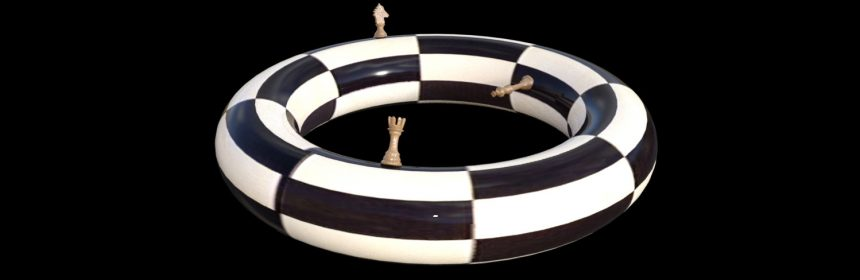Part VII Episode 2 of A Mathematical Chess Puzzle:

We are nearing the end, but still there is the tallest mountain to climb. We need to prove the SUPER QUEEN THEOREM, which says that not only is there a chess position $Q$ to the unguarded queen problem on the unit continuum board $B_c$ (where $c$ are is understood as all reals in the open unit interval (0,1)), but that in fact that solution satisfies the Super Queen property — which is to say, that not only is there exactly one queen on every rank and file (“Rook Maximal”), but that there is also exactly one queen on every diagonal (“Bishop maximal”). This is not possible on finite boards because we run out of places on diagonals to put the queens that don’t attack an existing queen by a rook move.

Note: we exclude the endpoints 0 and 1 from the intervals to remove the “degenerate” diagonals on the corners, and so ensure that all rows, columns and diagonals are of positive linear measure. If they were included, then only one of the two degenerate diagonals through (0,0) and (1,1) respectively could be admitted and the “super queen” property would not hold.

To prove this theorem, as mentioned in the previous post, we will need to use a technique called Transfinite Induction. This is a concept which extends a more conventional technique called Mathematical Induction. The general idea of regular induction in mathematics can be expressed using the metaphor of a ladder:

Mathematical Induction: If you can prove that

1. you can get on the first rung of a ladder, and
2. you can also prove for any N that
if you can get to rung $N$,
then you can also get to the next rung $N+1$,

then you have proven you can go all the way up the ladder.

This does not seem very deep, and in fact seems obvious, but it is a technique used in many parts of mathematics. For example, consider the theorem:

THEOREM: For every integer $n \ge 1$, the odd sum  $1 + 3 + 5 … (2n-1)$ is a square, and in fact

$$S_n = 1 + 3 + 5 … (2n-1) = n^2\tag{**}$$

For example, for $n=1$, $1 = 1^2$, for $n=2$, $1 + 3 = 4 = 2^2$, and so on.

PROOF: We shall use (regular) mathematical induction. The first step is to prove we can get on the “first rung”, so we look at $n=1$ and can see that indeed $1 = 1^2 = (2n-1)^2$. For the next step, suppose that formula (**) holds for a specific $n = N$, that is, for a specific $N$ we have:

$$S_N = 1 + 3 + 5 … (2N-1) = N^2\tag{***}$$

We need to show that (**) also holds for $n= N+1$. We can write

$$S_{N+1} = 1 + 3 + 5 … (2(N+1)-1)^2$$

$$= 1 + 3 + 5 … + (2N -1)^2 + (2(N+1)-1)^2$$

which by definition can be rewritten:

$$= S_N + (2(N+1)-1)^2$$

and by the assumption (***) we have

$$= N^2 + (2(N+1)-1)^2$$

which simplifies to

$$= N^2 + 2N + 1 = (N+1)^2$$

which is formula (**) with $N+1$ substituted for $n$. We have just proved the second part of induction, which is that if (**) is true for $N$ then it is also true for $N+1$, and so the theorem is proved. QED.

### The Rational Super Queen

As a first step toward proving the Super Queen Theorem on the transfinite board $B_c$, we will first prove the analogous theorem on the rational unit board $B_{\mathbb{Q}}$, where $\mathbb{Q}$ is understood as the set of all rational numbers between 0 and 1 exclusive.

Referring back to the previous article on the Rook, both the rational unit board and the omega board have a countable infinity of “squares”. And as in that case, what makes the situations different is that the omega board (whose coordinates are integers) has a discrete topology, while the rational numbers are dense, and no square has a unique square “next to” it.

It should be noted that when restricted to just rational points, the concept of rook and bishop lines restricted to $B_{\mathbb{Q}}$ makes sense. The reason is that for any two “rational” points $p,q \in B_{\mathbb{Q}}$,, and any two rook or bishop attack lines $\lambda_p,\lambda_q$ which pass through $p$ and $q$ respectively, that their intersection is either empty or another rational point $r \in B_{\mathbb{Q}}$. That is, you will never have two points on the board whose common points of attack are irrational points outside of $B_{\mathbb{Q}}$. (Proof left to the reader)

Let us define $\Lambda$ to be the set of all rook and bishops lines (ie, all Queen lines) in $B_{\mathbb{Q}}$. Then we have

The Rational Super Queen Theorem: There exists a queen position $Q$ on the rational unit board $B_{\mathbb{Q}}$, such that for every queen line $\lambda \in \Lambda$ the intersection $\lambda \cap Q$ has exactly one point $q \in B_{\mathbb{Q}}$. That is, every Queen attack line in $B_{\mathbb{Q}}$ is occupied by exactly one queen in the position $Q$.

We will prove this theorem by (regular) mathematical induction. To do this we will need some definitions and to prove some  lemmas first.

Definition: a set $S$ is Well Ordered if there is an operator (which we will call $\le$), such that for all $x,y,z \in S$ we have

1.  $x \le x$
2.  Either $x \le y$ or $y \le x$
3.  if $x \le y$ and $y \le z$ then $x \le z$
4.  if $x \le y$ and $y \le x$ then $x = y$
5.  (Well Ordering): For any subset $T \subset S$, there exists a “smallest” element $x \in T$, ie, so that $x \le y$ for all $y \in T$.

The first four conditions describe “total ordering”, while the fifth (important) condition is what makes a “well ordering”. The principle of Mathematical induction is equivalent to the statement that the natural numbers $\mathbb{N}$ is well ordered.

Example 1: The positive integers $\mathbb{N}$ are well-ordered with the usual numeric ordering $\le$.

Example 2: The set of all integers $\mathbb{Z}$ is NOT well-ordered under $\le$, because $\mathbb{Z}$ itself is a set having no smallest value (violating condition 5).

Theorem 1:  The rational points $(x,y) \in B_{\mathbb{Q}}$ have a well ordering (but not the usual one $\le$).

Proof: It is known that the positive rational numbers $\mathbb{Q}$ are countable; that is, that there exists a function mapping the natural numbers $\{1,2,3,…\}$ to $\mathbb{Q}$ in a 1-to-1 way. To see that, observe that they can all be represented as fractions $p/q$ in a square array whose x and y coordinates indicate the numerator and denominator (below). Note that fractions that are not in simplest terms (e.g. 2/2) are skipped.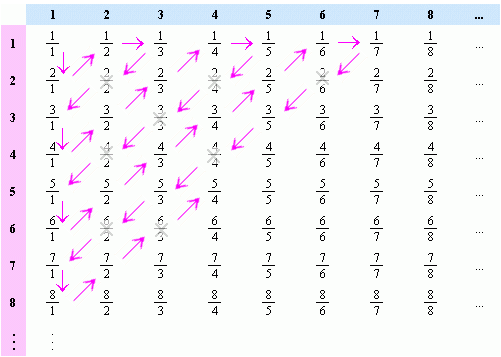As the arrows suggest, we can then “thread” the numbers onto a line, and number them by the order they appear, like this:

$$x_n = \frac{1}{1}, \frac{2}{1}, \frac{1}{2}, \frac{1}{3}, \frac{3}{1}, . . .$$

$$n = 1, 2, 3, 4, 5, . . .$$

This gives us a function $Q(n)$ which takes the integer $n$ to $x_n$. And so, we can define our new “ordering” (call it $\prec$) on the positive rationals by defining $x_n \prec x_m$ if and only if $n \le m$ in the usual sense. And this ordering is well-ordered, because for any subset $S$ of positive rationals, one simply considers the inverse set $S’ = Q^{-1}(S)$. SInce $S’$ is a subset of the positive integers, there must be a smallest one $m \in S’$, and by definition this is also the “smallest” in $S$, ordered by $\prec$.  QED

Theorem 3:  The rational points $(x,y) \in B_{\mathbb{Q}}$ have a well ordering.

Proof: We can follow a proof that parallels the one just given for Theorem 2. For we can use the inverse function $Q^{-1}(x)$ to map all rational points $(x,y)$ to non-negative integer points $(m,n)$, and by arranging them in a similar grid as above, “thread” the integer grid with a single integer line, and then use the inverse of this mapping to map the well-ordered integers to the rational points.  QED

Theorem 4:  The set $\Lambda$ of queen lines on the rational board is countable and can be well ordered.

Proof: Each (non-degenerate) queen line can be identified by a pair of distinct rational points $(x,y)$ and $(z,w)$ on the board which belong to it. The rational points are well ordered and (by the same arguments above), the pairs of such points also have a well-ordering.

Proof of The Rational Super Queen Theorem

Our goal is to prove that we can construct a position $Q = \{ q_0, q_1, . . . \}$ on the unit rational board, such that every queen line $\lambda \in \Lambda$ contains exactly one and only one element $q \in Q$.

By Theorem 4, the queen lines $\Lambda$ are countable. This means that we can assign an index $n$ to each of them which might as well be the integers 0,1,2…), which also gives them a well-ordering.

In addition, the points on the rational board itself is countable and has a well-ordering.

To prove the existence of a position $Q$ we shall use (regular) mathematical induction.

For step zero, we simply define $q_0 = b_0$ where $b_0$ is the first element in the well-ordering of the board. We also define $\Lambda_0$ to be the set of (four) queen lines which pass through $q_0$. Let us also define the set $Q_0 = \{ q_0 \}$.

For step 1, we consider the set of all queen lines which are not in $\Lambda_0$, and take the queen line $\lambda = \lambda_\alpha$ whose index $\alpha$ is the smallest among those. We then exclude from this $\lambda$ (considered as a set of points in the board) all points where it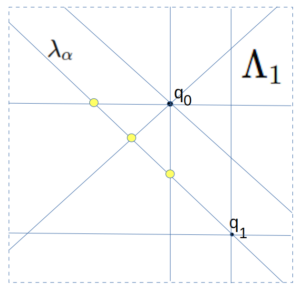intersects $\Lambda_0$ (shown here in yellow). This intersection is a finite set of points, and so we can pick for $q_1$ any other point on $\lambda$ not in this intersection. We then can define $\Lambda_1$ to be the set of all queen lines which pass through the set $Q_1 = \{ q_0, q_1 \}$. Note that $\Lambda_1 \supset \Lambda_0$ and is itself finite. We also note that any place where $\Lambda_0$ and $\Lambda_1$  share a point, those points are not in $Q_1$.

To generalize this last step inductively, for step $n+1$, we consider the set $S \subset \Lambda$ of all queen lines which are not in ${\Lambda_n}$, and among them choose a queen line $\lambda = \lambda_\alpha$ whose index $\alpha$ is the smallest within $S$. We can do this because ${\Lambda_n}$ is a finite set of queen lines, and so the remaining set is non-empty (in fact, countably infinite), and $\Lambda$ is well-ordered. We now consider the set of all intersections of $\lambda$ with lines in ${\Lambda_n}$. As before, this is a finite number of intersections and so we can choose for $q_{n+1}$ any point in the (infinite) queen line $\lambda$ not in this set, and define $\Lambda_{n+1}$ to be all queen lines passing through points $Q_{n+1} = Q_{n} \cup \{q_{n+1}\}$.

We now assert that (1) this tower of inclusive sets

$$\Lambda_0 \subset \Lambda_1 \subset \Lambda_2 . . .$$

is exhaustive, and its union will eventually include every single queen line $\lambda \in \Lambda$. In other words, the union is itself $\Lambda$. And furthermore, (2) the union of the tower of sets

$$Q_0 \subset Q_1 \subset Q_2 . . .$$

is our desired position $Q$ and that every member of $\Lambda$ meets $Q$ in exactly one point. The proof of this is below:

(1) To see that the $\Lambda_n$ tower is exhaustive, assume that this was not the case, and that there was some $\lambda$ that was missing from the tower, and so let us choose $\lambda_\beta$ to be the smallest of these missing ones in the well-ordering of $\Lambda$. But now consider how we constructed the set $\Lambda_{\beta + 1}$.  By construction, it was the union of $\Lambda_{\beta}$ with the  $\lambda_n$ with the smallest index $n$ which was not in $\Lambda_{\beta}$. But this means that $n$ must be greater than $\beta$, and so $\lambda_\beta$ should have been chosen at that step because it has a smaller index and is not in $\Lambda_{\beta}$.

(2) To see that every member $\lambda$ of $\Lambda$ meets $Q$ in at least one point, simply note that $\lambda$ must belong to $\Lambda_n$ for some smallest $n$ (since the union was exhaustive). But $\Lambda_n$ was defined to be all queen lines passing through $Q_n$, and so $\lambda$ must meet at least one element of $Q_n \subset Q$. But the points already chosen were selected from a set that excluded pairwise intersections of members of $Q_n$, and so $\lambda$ cannot possible meet any more than one element (as pairwise intersections were excluded). Hence $\lambda$ meets $Q_n$ at exactly one point, and by the construction, no later $Q_m$ sets meet $\lambda$ at all.

QED

This proof is remarkable, because nothing like it is possible with the finite boards. The thing which makes this work, is that at each step we are only removing a finite number of points from an infinite board, and so we can always find more in the remaining infinite sets to satisfy our conditions. Unlike the finite case, it did not matter whether any “queen line” was a rook or bishop style line. They were each treated equally in the set $\Lambda$ and the process of eliminating candidate points for the next step never exhausted the pool.

Having now proved the “Super Queen” solutions exists for the rational board, we are ready to pull out the big guns in the next post and deal with the Super Queen solution on the transfinite real board.

## A Chess Puzzle, Part VII Episode 1: The Fractal QueenPart VII Episode 1 of A Mathematical Chess Puzzle:

We have now come to the final (two-part) chapter of this two year journey, exploring the maximum “density” of various chess pieces on an infinite chess board, with the rule that no piece is attacking any other. The journey has taken us through many realms of mathematics, including geometry, set theory, group theory, differential calculus, real analysis, combinatorics, topology, measure theory, and transfinite numbers.

With that exhaustive list of mathematics, one would think that our last chess piece —- the Queen —- would have little more to offer us. After all, in terms of attacks, the queen is simply a Rook that can also attack like a Bishop, and so any position of “friendly” queens is also a position of friendly rooks and/or Bishops. In terms of set theory, the set of unguarded queen positions on a board is the intersection of unguarded Bishop and Rook positions.

But as we shall see, the Queen takes us on one final trip, through some of the deepest mathematics that we have yet encountered.

### Unguarded Queens on the Countable Board

There is one catch with the finite $N \times N$ board $B_N$, which is that while a maximal unguarded Rook  position consists of $N$ rooks, a maximal unguarded Bishop position will have $2N-2$ bishops, and so (on the finite boards at least) maximal unguarded queen solutions will not be maximal as bishops, merely “Rook maximal” (to coin a phrase).

There is a simple way in which one can take finite solutions to the unguarded queen problem and turn them into solutions on larger boards, and by extension to the countably infinite board $B_\omega$. We we show one such construction here.

For example, let’s start with a solution to the unguarded Queen problem on the 5-board $B_5$:

If we now go to the 25-board $B_{25}$, we can think of it as a $5 \times 5$ array of $B_5$ boards. So suppose we place one of the above solutions on each of the array locations corresponding to the queen solutions, like this:

it turns out that this is a Rook-maximal unguarded Queen position on the 25 board. It is not obvious that it is an unguarded Bishop position; even though it is clearly an unguarded rook position you have to verify that none of the queens in one block attack diagonally any other queens in the other blocks. Amazingly, none of them do. To see this, we only have to consider the diagonal attacks between the “blocks” of queens. As can be seen, when two such blocks are placed adjacent to each other, the queens in each block control separate diagonals from the other block:

In the exact same manner one can take this solution on $B_{25}$ and place five copies on a $B_{125}$ board to produce an unguarded queen solution there, and continue in with all larger boards that are powers of 5.

One can then extend this generally to the countably infinite board  $B_{\omega}$ by simply noting that each finite solution $Q_i$ contains all smaller solutions, and so you can simply take the union of all of them to cover $B_{\omega}$, that is. our position $Q_{\omega}$  is given by:

$$Q_{\omega} = \bigcup^{\infty}_{i=0} Q_i$$

To make this infinite solution explicit, we need to give for each horizontal integer coordinate $x = \{0,1,2 …\}$ the vertical location $y = \phi(x)$ where the queen in that column is located. There is a neat trick for doing this. We first explictly define the first five $y$ values of $\phi$ from $x=0$ to $x=4$:
$$\begin{cases} \phi(0) = 0 \\ \phi(1) = 2 \\ \phi(2) = 4 \\ \phi(3) = 1 \\ \phi(4) = 3 \\ \end{cases}\tag{PHI}$$
Note that $\phi$ is a permutation of the five digits $\{0,1,2,3,4\}$. We then define $\phi(x)$ for all other positive integers $x$ by writing its value in base 5, ie,
$$x_5 = d_1 d_2 … d_n,$$
where $d_i$ are base 5 digits, and then define
$$\phi(x)_5 = \phi(d_1) \phi(d_2) … \phi(d_n)$$
That is, we permute each of the input digits of $x$ by $\phi$ to get the base 5 representation of the output $y$. Note that this also shows that all rows contain a queen, because to find the column $x$ where a queen resides on row $y$, just write $y$ in base 5 and apply the inverse of $\phi$ on the digits to get $x$.

A close approximation of the infinite board $B_{\omega}$ solution can be seen below, with a scaled down version of the solution on the very large board $B_{625}$:

We should remark here that there is nothing magical about the number 5. We could just as easily have found another solution for the omega board using a $7 \times 7$ board queen solution as a template, such as this:

However, this approach does not always work. Not all 7-board solutions work for example, and no queen solutions on the 4-board work:

### The Troublesome Transfinite Queen

As with the Rook and Bishop, we now turn to unguarded queen problem on the board of the continuum $B_c$. Unlike the omega board whose “squares” are located at integer coordinate 0,1,2…, this board is transfinite, and has an uncountably infinite number of “squares” whose coordinates are real numbers. As with the Rook and Bishop, we will look strictly at the open bounded case where the board is the unit square (technically, that would be $B_I$, where $I$ is the open unit interval $I = (0,1) = \{ x: 0 \lt x\lt 1\}$. We have selected the open interval to eliminate the corner points and boundary.

As a naïve first attempt at constructing a solution, we might try the same trick as above, with an iterative process. The difference here, however, is that instead of a union of successively larger sets of discrete queens on the integer board, we create an infinite sequence of shrinking compact nested sets $Q_i, i=0,1,2,…$ where $Q_0 \supset Q_1 \supset Q_2 …$ and whose intersection forms the solution $Q$, that is,

$$Q = \bigcap^{\infty}_{i=0} Q_i$$

The first three iterations are shown below: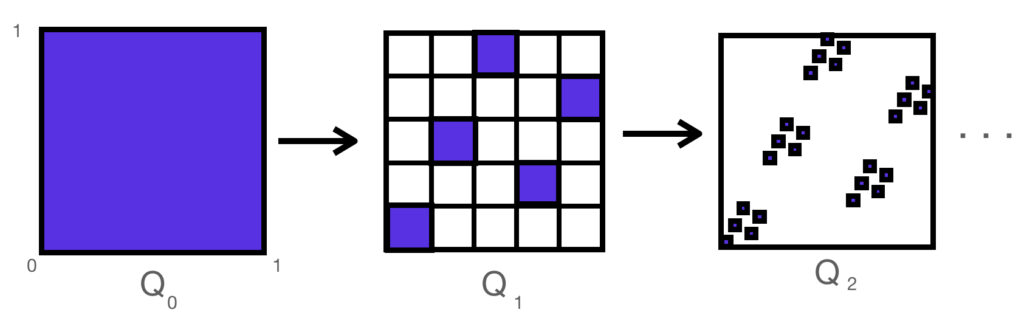And the resulting fractal set $Q$ looks very much like the board $B_{625}$ shown above. It can be shown that this set has a fractal dimension of 1, and a 1-dimension fractal measure of $\sqrt 2$

Now one would think that this “solution” $Q$ works, because at each iteration the cells do not “attack” each other as Queens. However, the actual “squares” on this transfinite board are points, so we need to make sure that there are no collisions in he limiting case.

### Failure!

… In fact, the solution $Q$ we have constructed is a failure.

Let’s first try to characterize the point set $Q$ mathematically, as we did in the case of the omega board.

In the omega board, whose squares had integer coordinates going off to infinity, we represented the values in Base 5, that is, multiples of powers of 5. In the case of the unit square, all coordinates are real numbers between 0 and 1, and we are dividing them by powers of 5.

We have the following theorem:

THEOREM: a point $(x,y) \in B_c$ is in $Q$ if and only if $x$ can be expressed as a Base 5 decimal, ie

$$x_5 = 0.d_1d_2d_3 ….$$

in such a way that the value $y$ can be expressed in Base 5 as

$$y_5 =0.\phi(d_1)\phi(d_2)\phi(d_3) …$$

And $\phi$ is the permutation on the digits $\{0,1,2,3,4\}$ defined by equation (PHI).

PROOF: The proof consists of observing that on the $nth$ iterative step $Q_n$ the points $(x,y)$ which are removed are exactly those where in their Base 5 representation the $nth$ digit of $y$ is not equal to $\phi(d_n)$. Conversely, if the $\phi()$ relationship holds for all digits of $x$ and $y$, then no iteration of $Q_n$ will eliminate the point $(x,y)$. QED

So if this is all good, what is the problem?

The problem is, there are many real numbers which can be expressed as infinite Base 5 decimals in two ways. In fact, any number whose Base 5 expansion terminates (ie, ends with 000…) has this property. For example, the (Base 10) value $x=0.2$ can be expressed (in Base 5) as either

$$x_5 = 0.100000000….$$

or

$$x_5 = 0.044444444….$$

(In the same way that in base ten 0.9999… = 1).

But if we apply $\phi()$ to these two decimal expansions we get two distinct values for $y$, ie

$$y_5 = 0.200000…$$

and

$$y_5 = 0.0333333…$$

…with the result that we have two distinct queens in the position $Q$ sharing the same $x$  value:

$$(x,y)_5 = (0.1, 0.2)_5 = (\frac{1}{5},\frac{2}{5})$$

and

$$(x,y)_5 = (0.0444…, 0.0333…)_5 = (\frac{1}{5},\frac{3}{20})$$

The two points can be seen here, along with (in red) many other queens in $Q$ which are mutally attacking, both by rook and by bishop moves:

And so we see that $Q$ is a total failure. Not only are there many members of this position that are hostile, but there are an uncountably many such failures!

As with the Rook and Bishop, the root difference between $B_{\omega}$ and $B_c$ has to do with topology. In the omega board, though infinite, it has a discrete topology with no limit points. In all of the cases with this continuum board, the queens which are attacking are limit points, belonging to members of the $Q_i$ sequence which bound the (rook or bishop) attack line from opposite sides of the line.

### An Unsolved Problem

Although I have tried a number of variations on this “fractal” approach, I have not found any explicit (fractal or otherwise) constructions of a queen position on the continuum board $B_c$ which is maximal unguarded one. That is, a set of points $Q \subset B_c$ defined by a closed-form computable function, such that for every horizontal and vertical line $\lambda$ intersecting $B_c$, there is exactly one point in $\lambda \cap Q$, and for every diagonal line, there is no more than one point in the intersection.

### A Solved Problem

As with many such questions about exotic properties of infinite point sets, it possible that no such closed form solution may even exist for the unguarded queens on the continuum board.

For example, a very similar problem to the unguarded queens is about the existence of “Two Point Sets”. That problem has been solved and can be stated like this:

TWO POINT SET THEOREM: There exists a set of points $T \subset \mathbb{R}^2$ such that every line in the plane contains exactly two distinct points of $T$.

This is a tantalizing statement, as it is about points and lines in the plane which satisfy a rule about which lines contains which points. And as with our unguarded queen problem, the quirky aspect of this (proven) theorem is that it only shows that a solution exists, but does not give any clue as to what it would look like.

In order to prove a theorem like this, however, requires some rather exotic techniques which depend on some deep hypotheses in set theory such as the Axiom of Choice and others. In particular, what is needed is a technique called Transfinite Induction.

This is a mathematical tool of which I had not been familiar during my younger days, and which most folks would have difficulty understanding at all, given that the word “transfinite” itself starts with Cantor’s discovery that there are sets which are so large that they are “uncountable” by the infinite set of numbers 1,2,3 etc.

### The Theorem

Using the techniques mentioned above, it turns out that not only will we be able to prove the existence of an unguarded queen solution as defined above, but an even stronger result, which has no equivalent statement on either the finite boards or even the countably infinite omega board.

We first begin with a definition:

DEFINITION: a chess position $Q \subset B$, satisfies the Super Queen property on $B$ if every horizontal, vertical AND (45-degree) diagonal line $\lambda$ intersecting $B$ contains exactly one member of $Q$.

Note that this is property is called super, because the property is not satisfied by any queen positions on the finite or countably infinite chess boards. On those boards, a queen position can be rook-maximally guarded positions, but not Bishop-maximally guarded. There will always be diagonals without queens controlling them.

The theorem we will prove in the next (and final) post on the topic will be the following:

SUPER QUEEN THEOREM: On the open continuum board $B = B_{I}$, where $I = (0,1) = \{ x: 0 \lt x\lt 1\}$, there exists a queen position $Q$ having the Super Queen property.

In other words, there is a position $Q$ on the board such that for every horizontal, vertical and diagonal line that intersects the board $B_{I}$, there is exactly one Queen on that line that belongs to $Q$ !

Stay tuned.

## A Chess Puzzle, Part VI: The BishopPart VI of A Mathematical Chess Puzzle:

We are now approaching the end of the story, with the final tale of the Fractal Queen approaching. But for now we must deal with with the Bishop, a close cousin to the Rook.

Recall that our puzzle revolves around the maximum “unguarded” positions of a chess piece on a board; in this case Bishops. In some sense, the Bishop can be thought of a Rook turned sideways, because both pieces can only move in two orthogonal directions, but the Bishop’s directions are along the 45 degree diagonals. The difference this makes though is that Bishops have a “color” while Rooks do not. Rooks can move to any square on the board while a Bishop on black squares can only attack other black squares.

This limited ability to only attack half of the squares that a Rook can, makes Bishops slightly “weaker”, and so it should not be surprise (as we showed in part I), that on an $n \times n$ board, we can place $2n – 2$ Bishops, giving them a density of roughly $\frac{2}{n}$ for large $n$, and asymptotically going to zero on the infinite board. So in some sense the friendly Bishop’s density on the board is roughly twice that of the slightly more powerful Rook.

But as with the rook, this is the two-dimensional density, while the unguarded Bishop positions clearly have a fractal dimension of one on the board. And as we saw with the Rook, once we go to the unit square board $\mathbb{B}_I$, with a continuum of “squares” at each real coordinate, the rigid shape of the solutions can loosen, so that instead of just the diagonal set with linear measure of $\sqrt{2}$ we can have a set of “friendly” Rooks on the board with 1-dimensional content of nearly 2:

and so, turning everything “sideways”, we can see that instead of a set of Bishops on the top and bottom row of the board with measure 2, we can stretch them toward each other, approaching a linear measure of almost $2\sqrt{2}$:

(Note that we have placed black and white circles in the corners to indicate that only the bottom corners are occupied with Bishops, which are in turn attacking the top corner locations). Again, as with the Rook solutions, there are no analogous version of these “continuum” solutions in the finite/discrete boards, as their topology is not dense.

Now one would think that the remaining piece — the Queen — would not be much different from the Rook or Bishop, as it simply has the capturing power of both. But as we will see, the problem suddenly become *very* different, and goes into some very deep and fascinating mathematics on the infinite boards.

## A Chess Puzzle, Part V: Rooks and TopologyPart V of A Mathematical Chess Puzzle:

### Review

We — that is, I — have been studying the (classic) “unguarded” chess puzzle, which is to say, how to pack as many of one chess piece on a chess board without any of the pieces attacking any others. But with the twist, that the board may be infinite, and possibly uncountably infinite. In the last post we saw that the set of maximal unguarded solutions for the Rook on the board $B_A$ (for any set $A$) had an interesting mathematical property, that they could be represented as the permutation group on the set $A$. In later posts we will see that the Queens also have some interesting properties, some of which can already be seen in our current focus on the Rook.

### What is Topology and Why does it Matter?

The glib definition of topology is “rubber sheet geometry”, which is to say, the study of what properties are preserved in an object even if they are made of rubber and can be twisted and stretched but not “torn”. For example, a solid ball and a solid cube can be deformed into each other just by stretching, but to deform a ball into a 1-holed doughnut you would need to puncture the ball to make the hole — which is not allowed.

One way in which a “topology” can be defined on a space is by specifying the way in which two points are “close” to each other, or whether two points can be “connected” by a continuous path.

We have been defining “chess boards” as two-dimensional things (denoted $B_A$) whose “squares” are identified with coordinates (x,y), where the x and y are in some subset $A$ of the real line $\mathbb{R}$. For example, the standard 8×8 chessboard we called $B_8$ uses the coordinates in the subset $\mathbb{Z}_8 = \{ 0, 1, 2, … 7 \}$ (though we could just as easily have taken {1, … 8} ). Among the other coordinate sets $A$ we could consider are the whole numbers, the rationals, the algebraic numbers, and the non-negative real numbers, or for that matter the whole real line.

### Topological Density

To see why questions about chess puzzles on various boards $B_A$ may have different answers depending on topology, consider that one of the properties of a subset of $\mathbb{R}$ is called density. A set $A \subseteq \mathbb{R}$ is said to be dense at a point $x \in A$ if for any other point $y \in A$ where $y \neq x$, there exists another point $z \in A$ strictly between them, ie, such that $(z-x)*(z-y) < 0$. The set A is everywhere dense if it is dense at every point of A, and nowhere dense (also called “discrete”) if it is not dense at any point of A.

For example, the whole numbers $\mathbb{W}$ is nowhere dense because there are no other integers between n and n+1. Meanwhile, the rationals $\mathbb{Q}$ are dense, because if $p$ and $q$ are two distinct rational numbers (ie, can be expressed as an integer fraction $m/n$), then it can be shown that $(p+q)/2$ is another rational strictly between them.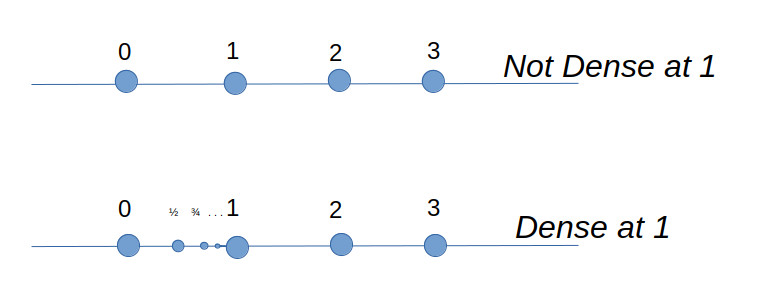And so, to see the reason why density is relevant for chess, simply note that the moves and attack regions for both the King, Pawn and Knight are defined by the squares *adjacent* to their current location, and on a board $B_A$ where $A$ is everywhere dense, there is no such thing as an adjacent square, because there is always another square in A in-between. In other words, the “unguarded” puzzle for Kings, Pawns and Knights does not make sense for dense sets $A$ such as rationals, algebraics, or reals. Meanwhile, because the attacks of Bishops, Rooks, and Queens is defined in terms of directions on the board (horizontal, vertical, diagonal), they have well-defined meanings even on everywhere dense boards.

### Connectedness

Strictly speaking, a set is topologically connected if it cannot be represented by a union of two disjoint sets. By that definition, a set like the integers or the rationals is nowhere connected. For example, the rationals can be represented as $\mathbb{Q} = \mathbb{Q}^+ \cup \mathbb{Q}^-$, where $\mathbb{Q}^+$ and $\mathbb{Q}^-$ are the sets of rationals greater and less than $\sqrt{2}$, respectively. These are both open sets because for any rational number $x \in \mathbb{Q}^+$, all other rationals (sufficiently close) to $x$ are also in the same set.

Nevertheless, we can still define “weaker” forms of connectedness on the rationals, in the sense that the closure of $\mathbb{Q} \subset \mathbb{R}$ is connected. There are also other more general definitions. A set is considered connected, intuitively, if you can get from any place in the set to any other by a “connected” path lying wholly within the set (whose definition needs to be made clear).

For example, suppose we take the set of whole numbers $\mathbb{W}$, and define that two elements $m, n \in \mathbb{W}$ to be “weakly connected” if they differ by no more than 1. So, 2 is connected to 3 and 1, as well as itself, but 2 is not connected to 4. More generally, we can say that two subsets A and B of $\mathbb{W}$ are weakly connected to each other, if there exists a member a in A and b in B such that a and b are connected. Finally, we can define a single subset $A \subseteq \mathbb{W}$ to be weakly connected, if for any two elements $a,b \in A$, there exists a finite sequence of elements $\{ a_i \} \subset A$ such that $a = a_0, a_n = b$, and where $a_i$ and $a_{i+1}$ are connected for all $i = 0…n-1$.

With this definition, we can see that the set $A = \{0,1,2,3,4\}$ is a weakly connected set, but that $A=\{0,1,3,4\}$ is not connected, because there is no way to to get from 1 to 3 by connected elements in $A$ (2 is missing and forms a gap).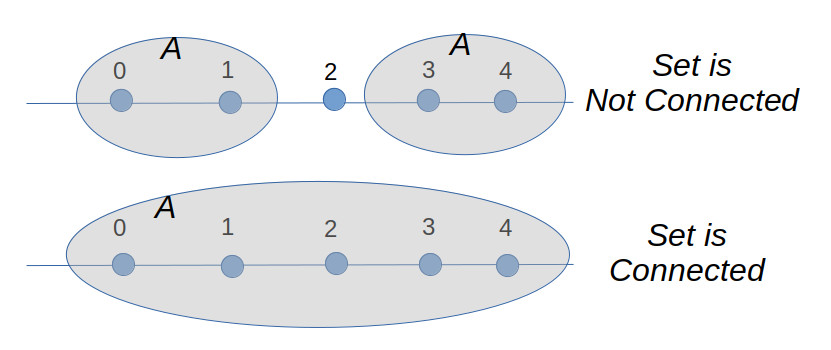The reason all this matters is that we will see that for the solutions to the unguarded rook problem, the topology of those solutions differs when we go from the infinite integer board $B_{\omega}$ to the infinite rational board $B_{\mathbb{Q^{+}}}$ or real board.

### The Topology of the Integer Board

Just as with the rationals, we can define “weakly connected” on the integer board $B_{\mathbb{W}}$, by first specifying that two squares a and b are “weakly connected” if they are a Kings move away from each other, that is, one square away horizontally, vertically, or diagonally. And by extension, we can say that any subset $A$ of $B_{\mathbb{W}}$, is connected, if any two members $a$ and $b$ of $A$ can reach each other, by a king moving strictly within the set $A$. From this point on, we will use “connected” to mean “weakly connected”, unless we say otherwise.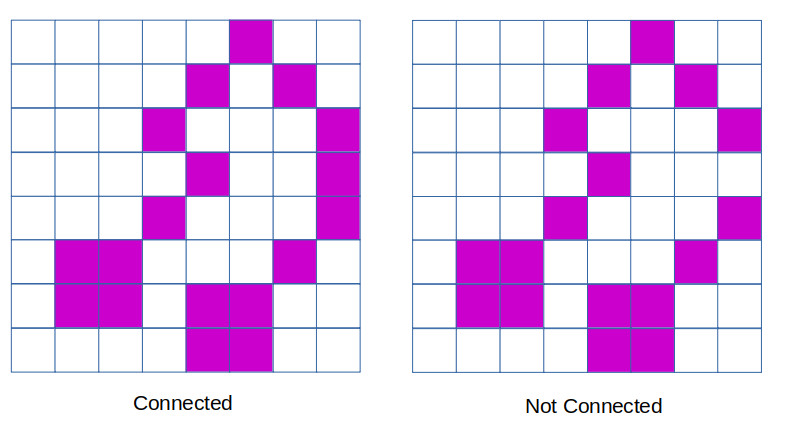Connected Rook Theorem 1: On any finite board $B_n$, the only connected maximal solutions to the unguarded rook problem are the two diagonal solutions, ie the graphs of the function $y=\phi(x)$, where

$$\phi(x) = x$$

or

$$\phi(x) = n – 1 -x$$

We first claim that if two squares in an unguarded rook solution are both connected to a third one, then all three must lie on the same diagonal (northwest or northeast). For, consider the case where square $a$ is connected to square $b$ along the north-east diagonal. We can go through and eliminate with an x all of the squares which are attacked by either $a$ or $b$. And what we see is that the only remaining square which a third rook can be placed and still be attached to $a$ is the lower-left corner.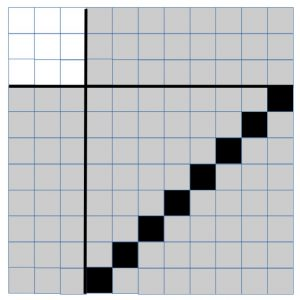By repeated application of this claim, what we have just shown is that all of the rooks in a connected component of an unguarded rook solution must lie on the same diagonal. Since this is a maximal unguarded solution, there must be a rook on the first row somewhere. We now claim that it must either be on the leftmost square (forming the northeastern diagonal solution), or else the rightmost square (forming the northwestern diagonal solution). For suppose that the rook is anywhere else along the bottom row, and that (without loss of generality) that it is part of a northeasterly diagonal.

Once again, by a process of eliminating all other squares under attack by the northeasterly diagonal line of rooks, we can see that this leaves a disconnected square in the upper left corner as the only available locations of the rooks that must occupy the remaining rows and columns. But none of these squares are connected to the northeast diagonal, and so a maximal unguarded solution cannot be constructed in this manner that is also connected.

QED

Connected Rook Theorem 2: On the infinite integer board $B_{\omega}$, the only connected maximal solution to the unguarded rook problem is the main diagonal solution, $\phi(x) = x$.

Proof: Clearly the above solution is maximal. Suppose that there is another maximal solution where there is a rook located at a position $P = (m,n)$, where (without loss of generality) we can assume $m > n > 0$. By eliminating all cells attacked by this rook, we now have a board segmented into four non-empty regions, (I, II, III, IV),  each of which would be disconnected from each of the others, save for their connection to the rook at $P$.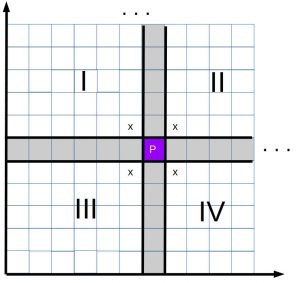By the same argument previously, either $P$ connects regions I to IV by the north-west diagonals, or else it connects regions II and III, by the northeast diagonals. If we choose to join regions II and III, then region III (including P) is an $m x n$ rectangle, in which we would have to fit $m$ rooks, even though we only have $n$ rows. This would force at least two rooks to occupy the same rows, which is not allowed. This leaves us with P joining up regions I and IV, by a northwest diagonal.  Now region I only has $m-1$ columns, while region IV has only $n-1$ rows, and so we have only a finite number of additional rooks we can add to this solution (region II not being connected to P). Thus neither option allows us to define a maximal solution to the unguarded rook problem, meaning that we must conclude that only the case where $m=n$ is valid.

QED.

### Unguarded Rooks on a Rational Board

While we could take the entire set $\mathbb{Q}$ of rational numbers, for our purposes it suffices to look at a much smaller but equivalent space, which is the unit interval of rational numbers, ie

$$\mathbb{Q}^* = \mathbb{Q} \cap [0,1]$$

The set of rationals is countably infinite, and so our board $B_{\mathbb{Q}^*}$ has the same number of “squares” as the infinite integer board. Nevertheless, the unguarded rook problem on this board has a surprising answer in store for us, given by the following theorem:

Connected Rook Theorem 3: There are an infinite number of connected maximal unguarded Rook solutions on the rational unit board $B_{\mathbb{Q}^*}$.

Comment: Just so you can see how on earth this could be possible, consider just one valid solution, defined on $\mathbb{Q}^*$ by the function:

$$\phi(x)= \begin{cases} \frac{x}{2}, & \text{if x \in [0, \frac{2}{3})} \\ 2x – 1, & \text{x \in [\frac{2}{3}, 1]} \end{cases}$$

The function $\phi(x)$ is a piecewise-linear function, which is a bijection on the set $\mathbb{Q}^*$.

The graph on $B_{\mathbb{Q}^*}$ shows that the placement of the rooks on the board looks like this: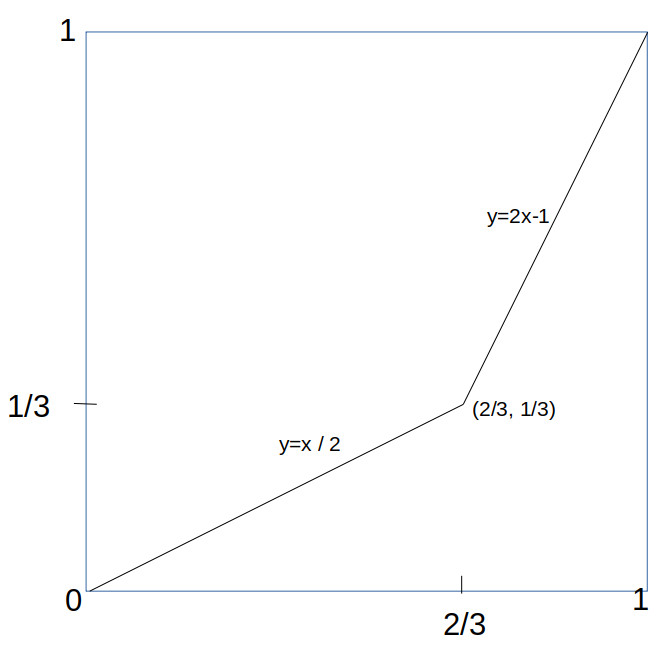what makes this work is that linear functions are invertible on the rational numbers, and that the rational numbers (unlike the integers) are *dense*. The same trick would not work on the integers, because you can’t apply y = x/2 to the odd integers and get another integer back.

Just to remind the reader of what this picture is about, that thin line is a chess position, consisting of a set of infinitely many rooks along that line, so an (infinite) magnification would reveal them, like this: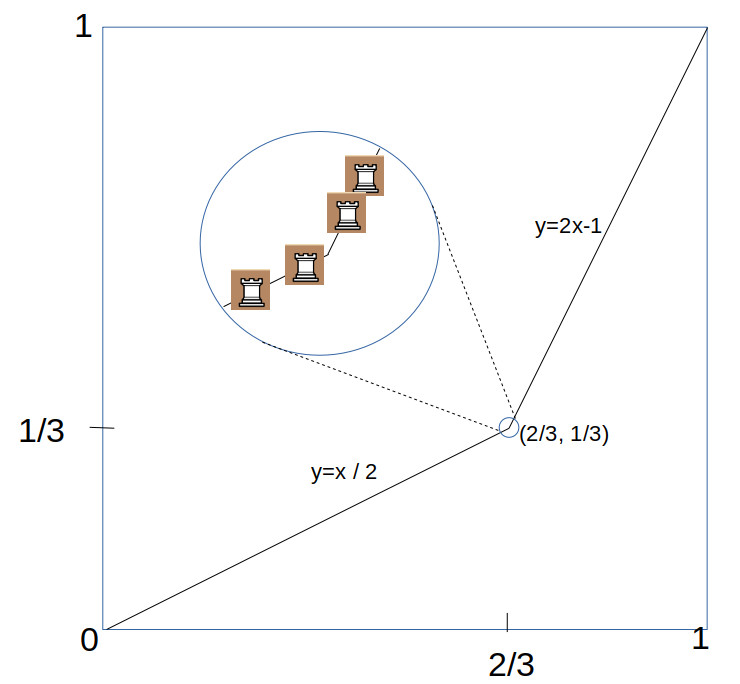This example is just one of many other such piecewise-linear functions which do the trick. The only requirement is that the functions must be strictly monotonic (increasing or descreasing) on the interval $\mathbb{Q}^*$, and (to ensure it is maximal), covers the entire interval, either by $\phi(0) = 0, \phi(1) = 1$ or else $\phi(0) = 1, \phi(1) = 0$.

It turns out that the number of such functions is infinite. This can be seen easily by just noting that there was nothing special about the bend at $x=2/3$, and you could create a similar function with a bend on any other rational number between zero and one, of which there are countably infinite many of them.

### Fractal Content

The (two-dimensional) density of the Rook solutions is zero, since lines have zero area. This is the case for all three pieces Rook, Bishop and Queen. But we can still compare the relative density of each of these pieces, by measuring the (one-dimensional) “fractal” content of the positions. For example, the fractal content of the solution shown above is simply the length of the two line segments, which is $2\sqrt{5}/3 \approx 0.75$. But we can actually do much better, by pushing the “corner” of the two lines toward the bottom right like this: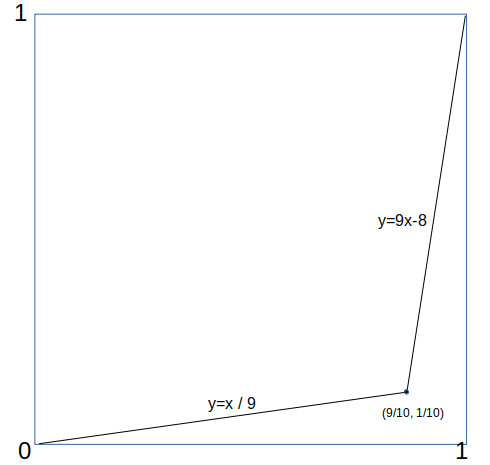in which case the fractal density is $\sqrt{82}/10 \approx 1.82$. As can be seen, by pushing further the rook solutions can attain fractal densities as close to 2 as we like.

### Uncountably Many Solutions

We showed above that there are an infinite number of connected maximal Rook solutions on the rational interval board $B_{\mathbb{Q}^*}$. But you can actually say a lot more; in fact the number of such solutions can be shown to be uncountably infinite, which is to say, there are at least as many of these solutions as there are real numbers (the infinity of the continuum) !

One way you can prove that the number of solutions is uncountable, is to construct a mapping between the (uncountable) set of all infinite decimals between 0 and 1, and a piecewise-linear function with rational coefficients, whose graph is a maximal connected solution of the unguarded Rook problem.

To do this, we first define for each digit from $i = 0 . . . 9$, a different piecewise linear function $f_i()$ on the unit square, simply by mapping the digits to different “bend locations” in the square (having rational coordinates):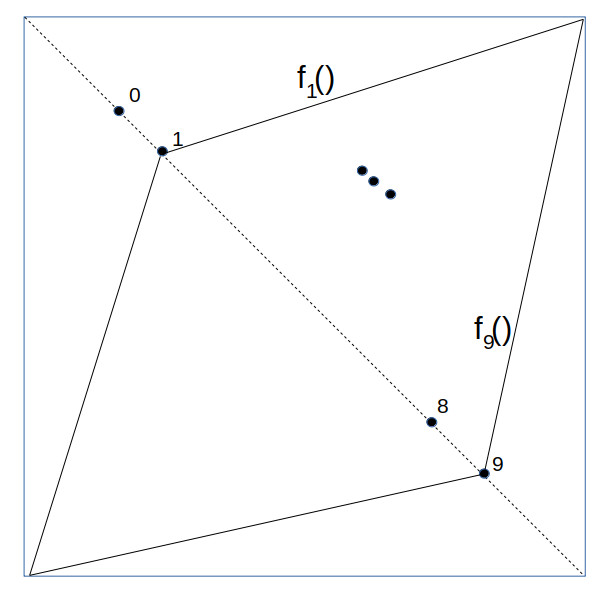Next, we take the unit square and along the main diagonal, lay out an infinite number of smaller squares, each half the size of the previous: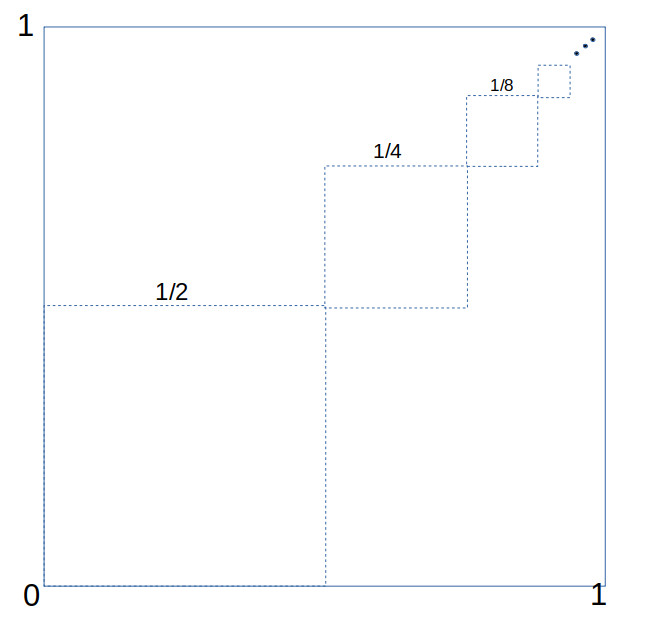We now show how to map each infinite decimal fraction $z$ (e.g. $z = 0.2739 . . .$ ) to a specific piecewise-linear function $F = F_z(x)$ on the unit square. For each of the smaller squares we have placed inside the unit square, we go through the decimal digits in $z$ and for each digit $d$ define the corresponding function $F_z(x)$ restricted to that range by $F_z(x) = f_d(x)$, corresponding to that digit $d$.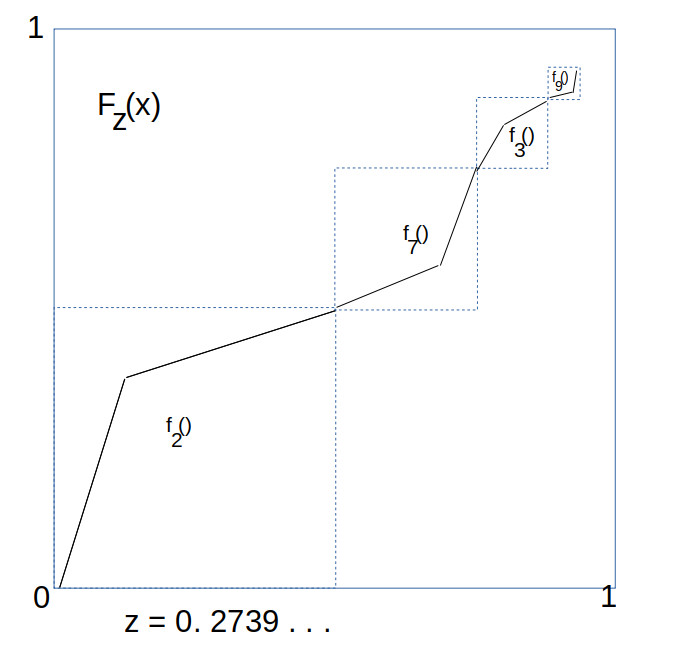As these infinite number of squares converge to the point $(1,1)$, we define $F_z(1) = 1$, which gives a complete definition on the whole unit interval. Since this mapping is injective, this means that the number of piecewise linear functions with rational coefficients is at least as large as the number of reals on the unit interval, which is uncountably infinite. This completes the proof.

So yes, there are a lot of them. And again, the reason this is so different from the integers has to do with the topological density of the sets.

Just to close the loop, if we look at the full real interval $I = [0,1] \subset \mathbb{R}$, it is actually much easier to construct topologically connected (in fact, compact) maximal unguarded Rook solutions. To construct such solutions, all that we require is that it be the graph of a function $y = \phi(x)$, where $\phi$ is a bijection on $[0,1]$ such that it is continuous and monotonically increasing or decreasing. That is, if $x > y$ then $\phi(x) > \phi(y)$. A simple set of examples of this are the powers of x, such as $\phi(x) = x^2, \phi(x) = x^3$, and so on.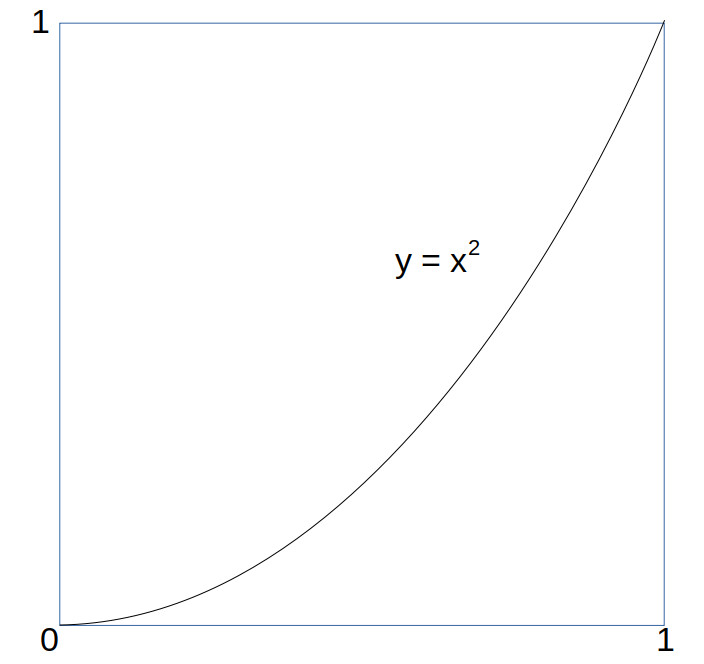As with the rational board case, with higher powers of x the curve begins to hug the bottom and right side of the board, with a curve length approaching 2. But can we do any better, or is 2 the largest 1 dimensional fractal content we can attain for connected unguarded rook solutions? The answer is given by the following theorem:

Fractal Content Rook Theorem: The path length of  a connected maximal unguarded Rook solution on the unit interval is not more than 2. That is, the length of any curve $y = \phi(x)$ on the unit interval $[0,1]$ where $\phi(x)$ is a continuous monotonically increasing function from 0 to 1 on the interval is less than or equal to 2.

Proof: We will need to use a little bit of calculus to do this. First, we parameterize the curve by $c(t) = (x(t),y(t))$ where the parameter $t$ ranges from 0 to 1, and

$$\begin{cases} x = x(t) = t \\ y = y(t) = \phi(x(t)) \end{cases}$$

From this we can compute the path length $s$ given by the integral (ie, sum):

$$s = \int_{t=0}^1 \frac{ds}{dt} dt$$

where $ds$ is the incremental increases in the path distance by $(dx,dy)$, given by

$$ds = \sqrt{dx^2 + dy^2}$$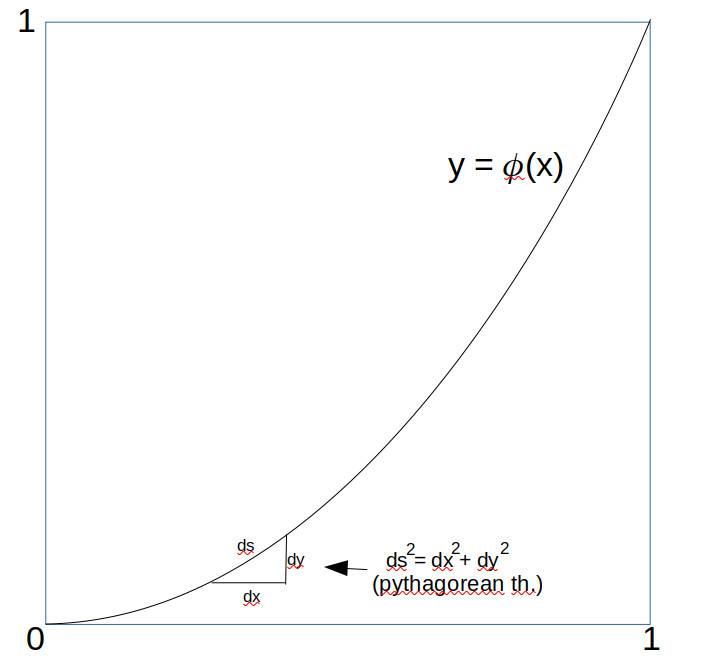For those unversed in calculus, think of $ds$ as just very small line segments into which the curve has been broken, and use Pythagorean Theorem). Now $dx/dt = 1$ since $x=t$, and $dy/dt \ge 0$, given that $y = \phi(x)$ is monotonically increasing. We can thus take advantage of the inequality

$$\sqrt{a^2 + b^2} \le \sqrt{a^2 +2ab + b^2} = (a + b)$$

when $a,b \ge 0$ to assert that (formally)

$$\frac{ds}{dt} = \sqrt{(\frac{dx}{dt})^2 + (\frac{dy}{dt})^2} \le \sqrt{(\frac{dx}{dt} + \frac{dy}{dt})^2} = \frac{dx}{dt} + \frac{dy}{dt}$$

and so we can conclude (since x(1) = 1 and y(1) = 1):

$$s \le \int_{t=0}^1 \frac{dx}{dt} + \int_{t=0}^1\frac{dy}{dt}dt = 1 + 1 = 2$$

which was to be proved. QED

We have now pretty much exhausted the study of unguarded Rooks, so it’s time to touch base briefly on the Bishop, before we move on (at last) to the unguarded Queens.

## Sedona Journal – 2020### Preface

With the prospect of lengthy election-related noise in front of us, Gigi and I decided to take a week-long vacation from the world and head down to Sedona, Arizona, far from technology, news, internet, phone, or other channels by which the world would tell us more than we want to know.

### Phoenix, Arizona

Our first leg of the trip would be a drive down to Phoenix Arizona to visit my younger brother John and his wife Sheri for the weekend. On Sunday we plan on heading north to Sedona for the rest of the trip.

#### Friday, October 30, 2020

Sunny, warm

We drove down the 89A along the North Rim of the Grand Canyon, and from there straight down the 17 into Phoenix and John and Sheri’s house. They gave us a tour of their house; they had moved back to Arizona from Sacramento, where John had been working for a while.

#### Saturday, October 31, 2020

Sunny, shorts weather

Desert Botanical Garden

In the morning we visited the Desert Botanical Garden, whose exhibits were accented by large colorful plastic animals.

Barking Bar

Later that day we took bikes over to the “canal” and stopped at the O.H.S.O “Barking Bar” Brewery which served good beer, nachos, breakfast burritos and other fare. Also Mimosas. The bikes in the background have fans in the wheels. Camelback Mountain in the distance.

This was a dog-friendly venue and many dogs hung out with their masters and had lively conversations.

Arizona Falls

After brunch we rode further down the canal to Arizona Falls, a dam whose design was inspired by Frank Lloyd Wright, and includes poetry about water and life.

Halloween and Fossils

That evening was Halloween, and we sat out front and ate chili while we handed out candy to the neighborhood kids. I noticed that John had framed a set of fossils, including the trilobite that our grandfather Norvis (“Nick”) Edwards had found during a road construction job, and sent to us.

### Sedona, Arizona

#### Sunday, November 1, 2020

##### Corn Maze (“Maize Maze”)

John and Sheri joined us on our way out of Phoenix at Tolmachoff Farms, to explore a Corn Maze. The maze folks gave us a blank map of the maze, which we could fill out with patches of the maze map that we may run across along the way. As a mathematician, my brief glance at the overview map told me that we could “solve” the maze using the simple “right hand rule” (run your right hand along the wall and follow it where ever it goes). We spotted several of the patches (and some riddles) on our path to the exit, but did not find all the patches. So instead of going out the exit I followed the right hand back into the maze, which took us to other segments that (mostly) recovered the other patches. Fun!

Postscript to Maze: After returning home, I suddenly noticed that the shape of the maze was a pickup truck. I pointed this out to Gigi, who replied that she and Sheri noticed that it was a truck all along and were even commenting on being along the “fender” etc but (me being obsessed with solving the maze) had never noticed this obvious fact.

##### Arrival at Briar Patch Inn

Sunny, cool but pleasant

After leaving Phoenix we drove up to Sedona and checked in at the Briar Patch Inn bed and breakfast. We had reserved their “Heron” cabin, which had a secluded patio and was next to Oak Creek. We were married at the Briar Patch back in 2002, and when visiting we usually stay in the Casa Piedra cabin, but it was booked. The weather was pleasant, sunny but cool. By this time I had put my phone on Airplane mode, and there are no phones or TV’s in the cabins. The world outside was going away.

The Briar Patch Inn has a dozen or so small rustic cabins scattered about the tree-lined grounds, some large enough for whole families with full kitchens, others just enough for one person with few needs but privacy.

The grounds surround a grass-lined lawn where two sheep graze. When we first came to the Briar Patch, their names were Woolly and Bully. They have since moved to greener pastures, and we are now hosted by two sheep named Rosie and Posie.

The swimming hole is at a place where the creek slows down, bounded on each side by small cascades. It is a bit too cold now to swim in, but is very pretty and peaceful.We settled in for the night and lit a fire in the fireplace, with a large stack of books to read in cozy settings.

#### Monday, November 2, 2020

We drove out to “Montezuma’s Castle” and “Montezuma’s Well”, both ancient anasazi settlements notable for having nothing to do with Montezuma (who lived centuries later and never came through here). We reconnected to the world just long enough to get word about our cat Desert Kitty and our dog Blue. Kitty had been feeling bad when we left, not eating, but we got good news that he (whom our cat-sitter renamed “D-Cat”) was eating again.  At least now I would sleep better.

#### Tuesday, November 3, 2020

Cloudy, thunder and lightning. Rain.

Election Day in the US. We are offline.

One way or another, the good news is that after realizing this object was not functional, in the process of making it so I found that I learned a lot more about sextants that I ever would have, had I bought a truly functional precision instrument (for $200 more) in the first place. So let’s get to work. ## The Sextant Though they look complicated, the simple idea behind a sextant (or quadrant, octant etc) is just to measure the angle between two things in the sky, either two bodies (like the moon and Regulus), or between one body (Polaris or the sun) and the horizon. This is easy to do on land, but at sea with everything moving it is difficult. The clever idea (which it appears Newton had first) is to use two mirrors (actually, one and a half), in such a way that the two objects you are measuring can be brought “next” to each other optically by adjusting one of the mirrors. Once done, it is then just a matter of precisely measuring the angle the movable mirror was rotated. This nice diagram below (gleefully stolen from Wikipedia) shows how to get the (elevation) angle of the sun above the horizon: While we are on the topic, we should show the proper names of all the main components of the sextant: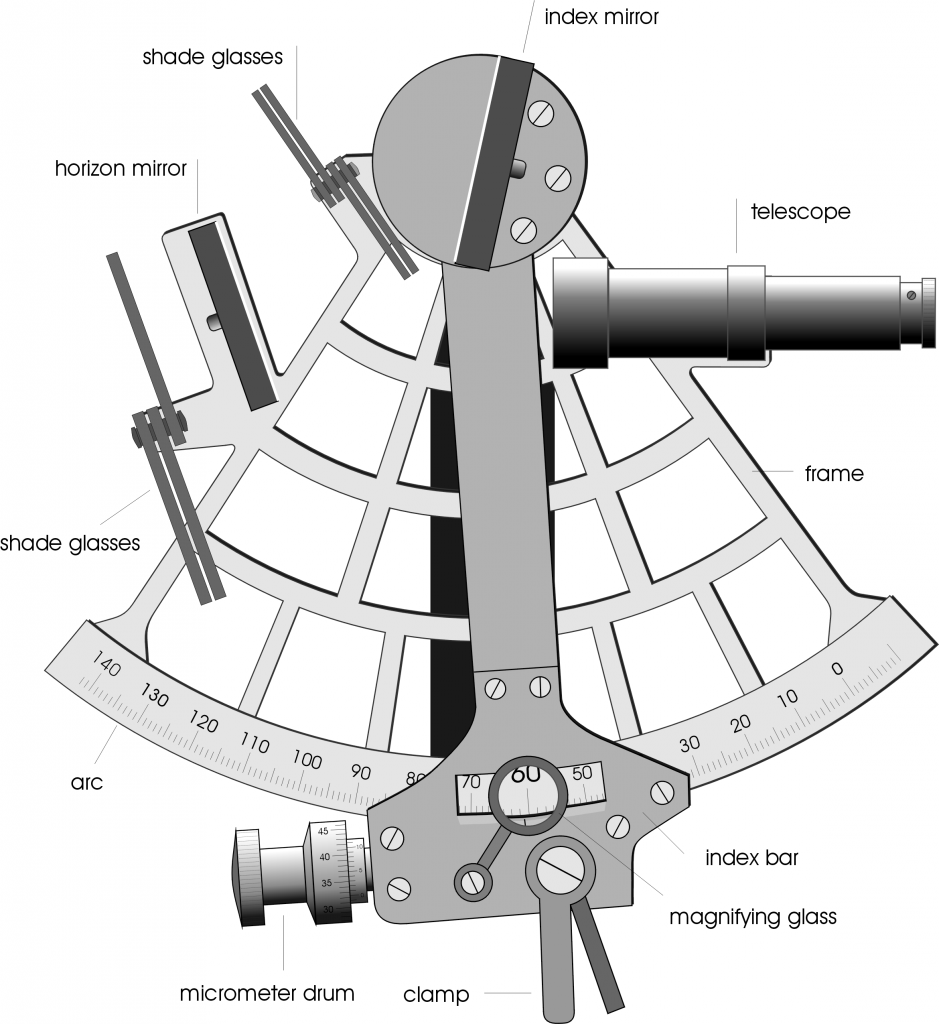The main elements are the frame, which is the 60 degree wedge that forms the base of the sextant. There is usually a handle on the other side of the frame so you can hold it. Along the outside of the frame is the arc, which has degree markings, starting from zero on the right. The frame also holds the fixed “horizon mirror“, which is only half-mirror, half clear glass. The movable arm is the index bar, which has a pointer (the index) that points to the angle on the arc. The “index mirror” is fixed to the index arm, and is a full mirror that rotates on a pivot and brings the second object into view. The shade glasses are deployed for shooting the sun, and prevent you from going blind. The drum allows you to do fine adjustment of the index arm, which whose angle on the arc you can see with the magnifying glass. Finally, the telescope is a tiny low-powered telescope which allows you to get a good look at the objects whose angle you are measuring. Here is an oblique view of my sextant, lying on its side. Ordinarily the geared arc is pointing down toward the earth. You can see that the horizon mirror is clear on the left side, and mirrored only on the right. So when you hold the sextant with the the telescope pointing to the horizon, the left side is looking straight ahead, at the horizon. Meanwhile, the right side is reflecting light from the index mirror, which is coming in at some angle above the horizon (indicated by the index arm).Here for example is the sextant with the index arm angle set to zero. This setting should allow the light from the horizon to bounce off the two mirrors and come into the little telescope at zero degrees. In other words, the view in both the left and right half of the horizon mirror should match.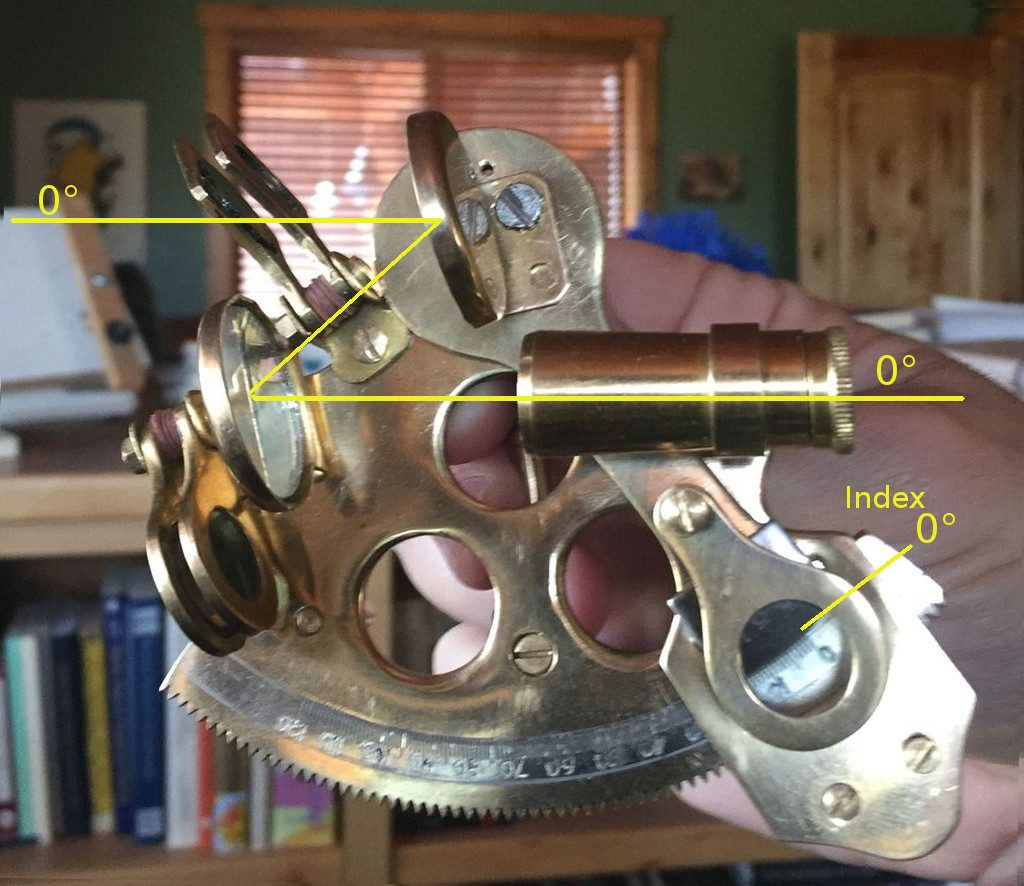## The Geometry The first geometrical question to address is, how does changing the angle θ of the index arm affect the angle β of the light coming in from the index mirror? Intuition suggests that since there are two mirrors, the angle will be doubled. Indeed, the rule is:  The angles on the arc of the frame should be marked like a protractor, but with the angular values doubled. Of course (for me) this requires proof. We will need to draw a diagram: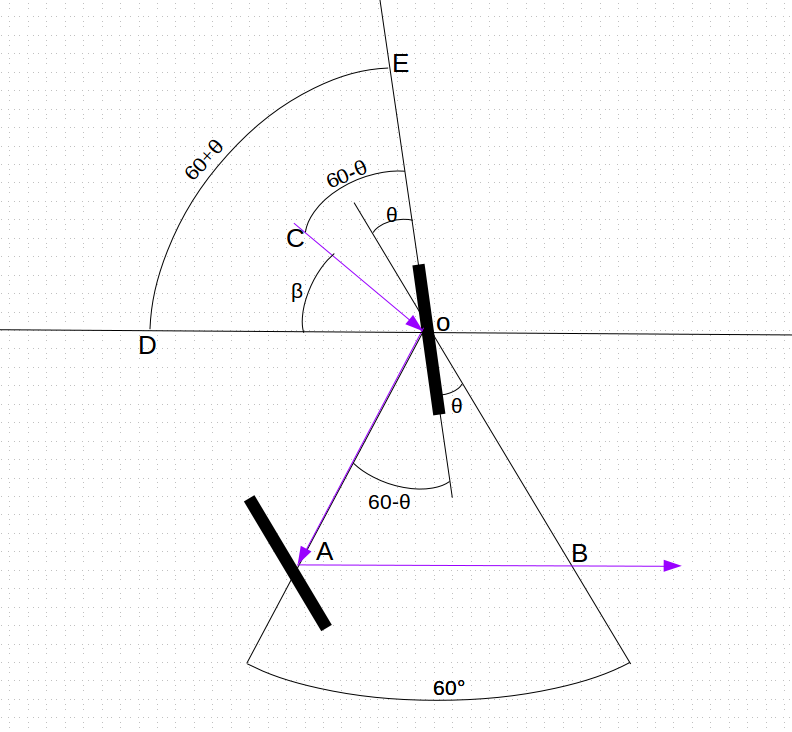The lines in blue show the path of light coming in along line CO, reflecting off the index mirror at O, continuing along line OA, and then reflecting off the horizon mirror at A, finishing along AB to the telescope. Let’s assume the index mirror makes an angle of θ with the line OB, and so the angle between the ray of light OA with the mirror at O must be 60°-θ. Now light bounces off of mirrors at the same angle they came in, so the incoming ray of light CO must also form the angle COE which is equal to 60°-θ. Finally, the index mirror forms an angle EOD with the horizontal line OD of 60° + θ, meaning that the residual angle β we seek is the angle EOD minus EOC, that is, β = EOD – EOC = (60° + θ) – (60° – θ) = 2θ so β = 2θ. That is, the angles marked on the arc, in order to properly represent the incoming angle β of light on the index mirror, must be a value of exactly twice the actual angle formed by the index arm at that point from the zero mark. ## The Reformation It didn’t take long for me to discover that my shiny new$15 sextant was not really functional as-is. It needed work. So the first thing I did of course (as is my nature) was to take the whole darn thing apart.### Issue #1: Frame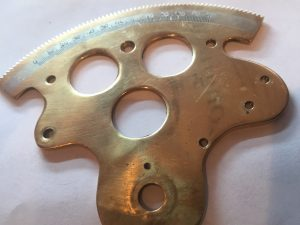The largest and most important component is the Frame — the large flat bit with the round gear-teeth around the edge. This serves as the “optical bench” upon which all the components are mounted. In addition, the gearing must be uniform, and the markings on the Arc calibrated, so that precise angular measurements can be made.

The first problem was that the frame was not flat. As many of the components are mirrors which must be aligned in 3 dimensions, the subtle bends in the frame would throw off any measurement. With all the pieces now removed, I was able to flatten out the frame.

The second problem was that the markings on the arc were clearly not precise. One clue may be found in the numbers, which on close inspection seem to have been crudely carved in by hand with a Dremel or similar tool. This is not a problem that can be resolved, short of recasting the whole thing.### Issue #2: The Index Mirror

The first thing to note about the correctly made sextants in the previous section is that the index mirror is not aligned with the axis of the index arm, but slightly off, about 15 degrees. The one I got by comparison was not like that, and its mirror was lined up exactly with the index arm like this: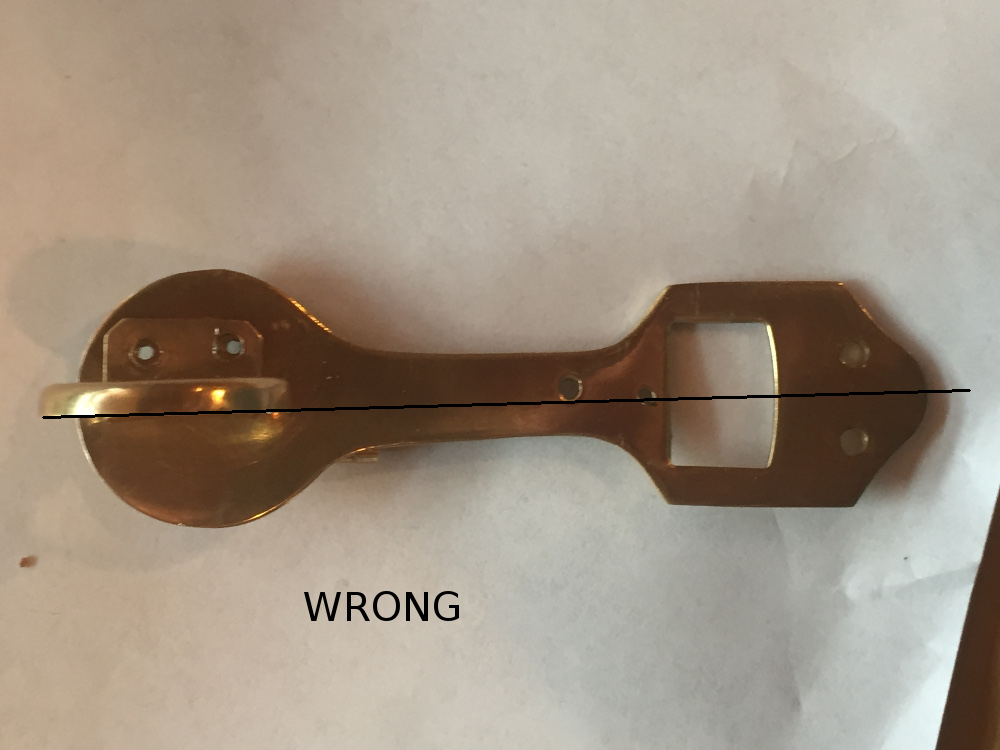It turns out that this is wrong, or at least not very good or practical design. In fact, what it should look like is this: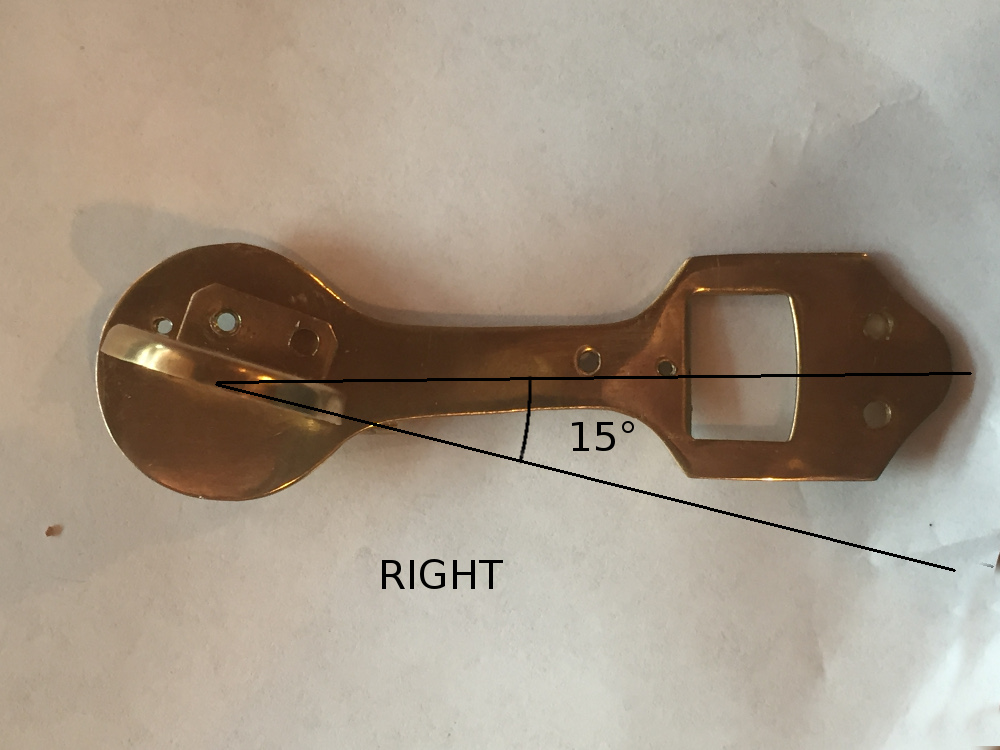Now the actual angle does not need to be 15°, but should be around there. The rule here is a bit more heuristic and goes like this:

 The index mirror should not be in line with the index arm, but offset by an amount greater than zero but less than 30°. 15° is close to ideal.

So what’s going on here? This is a mixture of mathematics and practical engineering.

Let’s parameterize this situation, and define a sextant whose index mirror is off-axis by an angle of δ a δ-Sextant. By this definition, what I bought is a 0°-Sextant, while the ones in the cartoon diagrams appear to be 15°-Sextants.

Okay, so what is so bad about my 0°-Sextant? Well, for looking at objects near the horizon (ie, where the index arm is near 0°), there is nothing really wrong at all and it works fine. I’m not familiar with the original design considerations, but two big factors have to do with the positioning of the horizon mirror, and the light-gathering ability of the index mirror. So let’s consider the horizon mirror first. Here is the geometry of the general δ-Sextant, with the index arm set at zero (so we are looking at the horizon):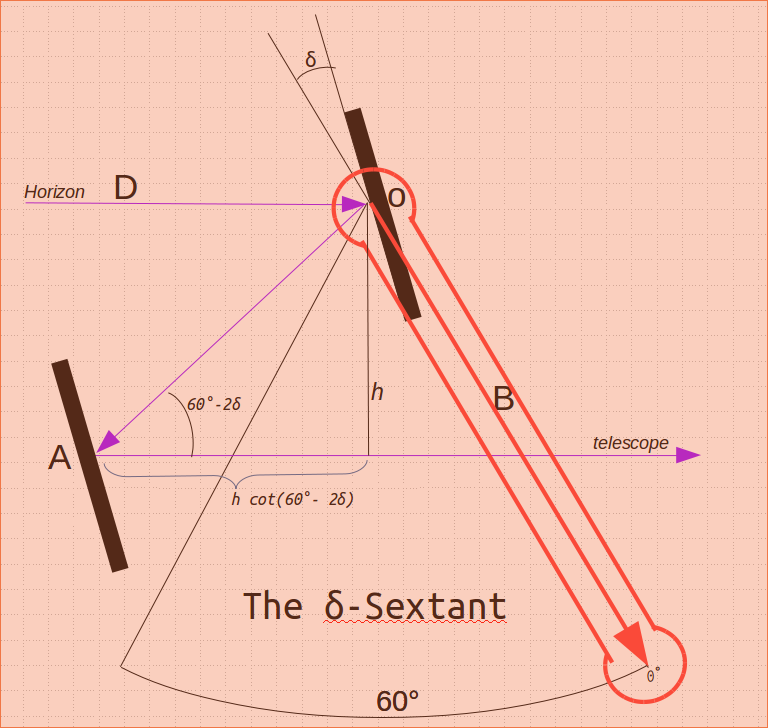Now by the same argument as before, the successive reflections of the ray of light coming in from the horizon form an angle of 60° -2δ away from the horizontal. So in order to the light to drop down a height of h (so that it can reach the telescope), the horizon mirror must be set back by h*cot(60° -2δ) from the center of the sextant. As we increase δ from 0 to 30 the cotangent approaches infinity, making the mirror position increasingly impractical.

Therefore, taking a mid-point value of δ=15° would put the mirror in a practical location, much less than infinity. The mirror was mounted on the index arm with two small screws. I drilled two new holes for the screws and used a tap-and-die kit to thread the holes for the screws. The new mount for the mirror brought it close to the required 15 degree orientation.

### Issue #3: Index Gear “Drum”

The index gear “drum” as depicted in the good diagram is a very precise worm-and-gear arrangement, with a “micrometer” fine-tuning for getting fractional degree measurements.The knob is supposed to engage the gear teeth in the frame, and rotate the movable index arm (on which the index mirror is mounted). However, instead of using a worm-and-gear mechanism, it has a “direct drive”, in which the knob turns a wheel gear that meshes with the frame.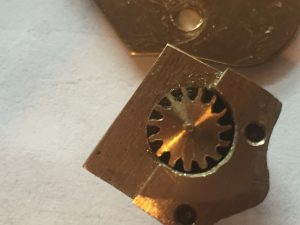On close inspection, it was found that this gear must have been made by drilling out each of the gear teeth, and manually filing them down. The width and separation of the teeth have a visibly discernable variation, of about 5% or so. This bit is of the “juggling dog” variety, which is to say the amazing thing is not that it does its job well, but that it does it at all. Indeed, this gear tended to jam up at certain parts of the arc, and so required some additional filing just to get it to juggle at all.

### Issue #4: Vernier Scale

In place of the high-precision worm-gear/micrometer, this sextant uses a “Vernier Scale“, which is admittedly a very clever device that was invented in ancient China (but named after French mathematician Pierre Vernier) to extract more precision out of otherwise crude devices. The general idea is to have a second scale that is just slightly smaller (9/10ths) than the base scale. This makes the smaller scale lines rarely line up with the base scale, except at one marking, which indicates how many tenths of a marking need to be added to the base reading:

Here is what our vernier scale looks like (bolted to the index arm):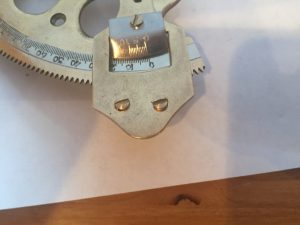There is just one problem with our Vernier. The scale is not 9/10’s of the base, but 10/10ths: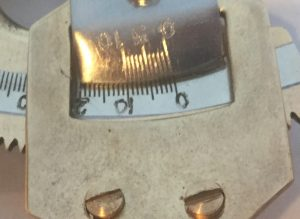In other words, as a Vernier scale, it is totally useless. It is most likely that the poor starving artisan in Bangalore was just told to copy another copy and assumed the scales were supposed to match. There is no way to fix this. But as the gears that drive the index arm along the arc are themselves only accurate to about 5%, the additional precision that would be provided by the Vernier is pointless. At least it has a 0 degree line, with which I can line up the 0 on the arc and calibrate zero-degree separations.

### Issue #5: Sun Filters

While sextants are often used at night to measure distances between stars, they are also used to calculate the Sun’s altitude above the horizon (at noon for example). For this reason, sextants come with sun filters to prevent injury to the eye. The sun filters on this sextant are faintly colored glass, and should NEVER be used for any reason. Hopefully, nobody ever has used them. They are, like the rest of the sextant, purely decorative. The only fix is to replace with actual solar filters, or remove them altogether.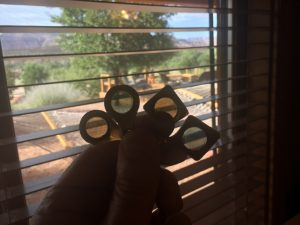### Issue #6: Telescope

To its credit, the telescope is not really a problem. It even appears to have a very slight magnification, and is properly aligned with the fixed horizon mirror.

### Issue #7: The horizon mirror

The horizon mirror, ideally, is split down the middle, with only the right half mirrored and the left half transparent, allowing the direct forward view to come through. Unfortunately, the mirror was glued in slightly at an angle, so that the vertical line between the mirrored and transparent sides is tilted by about 5 degrees. I did not want to risk breaking the mirror so left it alone. It was also offset vertically from the index mirror, and so added spacers to raise it up a bit.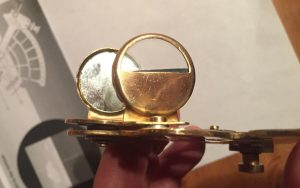### Issue #8: The Handle and Horizon bars

The back of the sextant has a vertically oriented handle, along with two posts which when aligned should match the horizon (when computing the sun’s altitude):The vertical handle was not exactly vertical, and so I inserted a spacer to adjust the alignment:### Issue #9: Magnifying lens

The magnifying lens, intended to magnify the vernier and base scales for easy reading of the angle, doesn’t magnify. It appears to be an optically flat piece of glass, identical to the “sun filters”. I removed it as it only gets in the way.

## Conclusion

This was a pretty but functionally useless toy when it arrived. After two weeks and a number of visits to Ace Hardware, it was almost serviceable enough to calculate separations to about 1 degree. I would not depend on it to save my life.

## The Planet Uranus in OppositionNote: I hope to update this piece when I catch Uranus at the turning point, August 2018. As of right now we are in thunderstorms, so it may take a while.

## Okay Let’s Cut to the Chase

Here is an animated GIF of two astrophotographs I took of the planet Uranus from my house in Virgin, Utah. The first one was taken on October 24, 2017 around 2:00 am, the next on November 19,2017 at 10pm (click on the image for full-size animated versions). See if you can spot Uranus. In the course of that month it has moved a bit, near the center of the image, so you should be able to see a blue-green dot jumping back and forth.

If you still can’t catch it, here is an annotated versions, with labels and stuff (again, click on the images for the full screen version):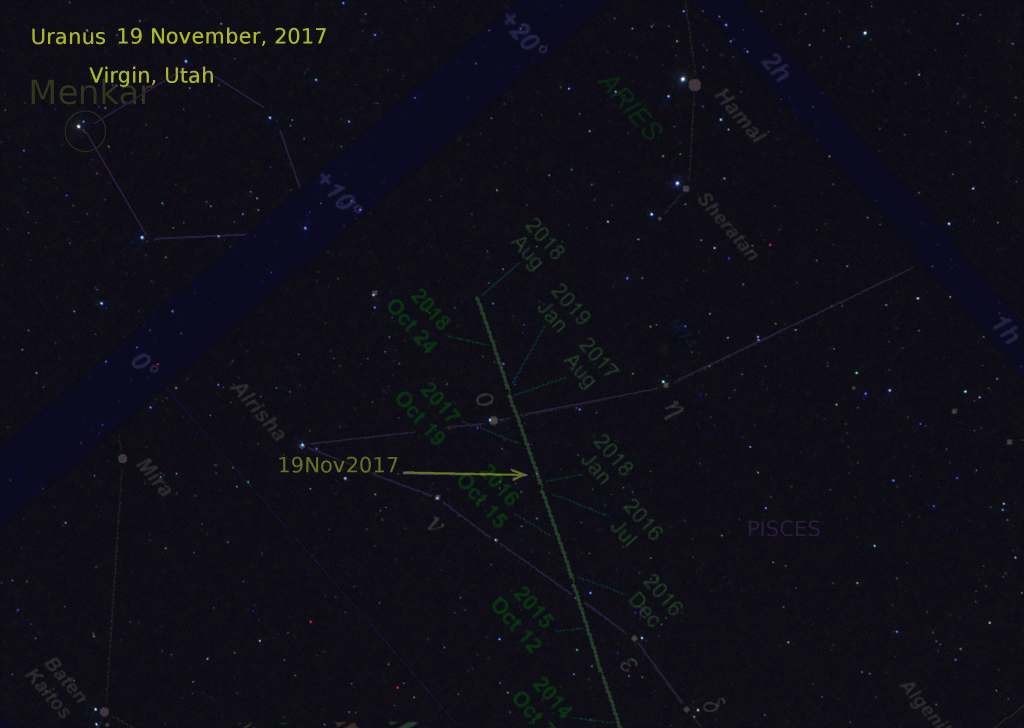In addition to the dated labels, I have put in some graphics showing the constellation Pisces, as well as a chart, showing where computer models say Uranus should be in that part of the sky, for various dates between 2016 and 2020. I had to pull all of these other things in, just to convince myself that I really caught the planet, and not just a random earth satellite or other transient object.

It has taken me quite a bit of work to get to this final product, of which I am quite proud, and happy that it came out so cleanly, riding exactly along those predicted lines. In August of 2018 (now) I hope to capture that endpoint of maximum extent. The rest of this blog piece is the retelling of the story of this image, along with the occasional digressions into the geometry of the whole thing.

Here is a picture of the planet Uranus, taken by the Voyager II spacecraft in 1986.

By the time I came to work at the NASA Jet Propulsion labs in ’87 the Voyager II probe had already passed by Uranus and was approaching Neptune, so I never got a chance to see these “live” images coming in. It’s not much to look at, and is best described as a large ice ball (unlike Saturn or Jupiter which are mostly gaseous). Even with a really good earthbound 8″ telescope, you’re not going to see much more than a fuzzy dot.

Though it had been seen before (even in ancient times), the object was not identified as a planet until it was observed and reported by William Herschel in 1781, who thought it might be a comet. However, after reporting it to the Astronomer Royal Nevil Maskelyne (who figures prominently in the quest to measure Longitude), Maskelyne concluded that it was probably a planet.

Other than its name (being the only one in the solar system based on the original Greek gods, and not the later Latin names of the Roman gods), Uranus is notable for having its rotational axis nearly horizontal to the orbital plane, so that for half the Uranian year (about 45 earth years) the “north” pole is in perpetual day, and the other half the year is perpetual night.

## Uranus in Opposition

What started all this for me was the announcement last month that the planet Uranus was in what they call “opposition“, meaning that it was on the opposite side of the celestial sphere (as seen from Earth) as the Sun. From the Sun’s perspective, this means that the Earth and Uranus are on the same side of the Sun, and typically on closest approach to each other:

The news in social media suggested that it would be so close that “it could be seen with the naked eye.” That sounded like hogwash to me, as there have been a lot of viral bogus memes around about being able to see things like the rings of Saturn and such.

Having now tracked down the planet, I can attest that — technically — it would be possible for a young person with excellent sharp eyesight to see the planet Uranus without binoculars … if they knew exactly where to look, and gazed at it out of the corner of their eye, and in a place (like where I live) with extremely dark skies and no cities nearby, but only on a cool clear night. But otherwise, forget about it.

## The Plan

Anyway, with the announcement of the opposition of Uranus in October I decided that this was a good opportunity to do some amateur astronomy and try to capture Uranus with some very low-tech equipment, which is a Nikon D-5000 camera mounted on a crude “Barn Door” equatorial mount. Using this mount, I can take long-exposures of up to 15 or 20 minutes, without smearing of the stars due to earth’s rotation.

The Barn Door mount is a clever contraption which anybody can build with $20 of parts from Ace Hardware or Walmart. The idea is simple, you just have two boards attached with a hinge, one board fixed to a tripod. You line up the hinge with Polaris (the north start), and mount the camera to the board that moves. But before setting up my rig, I first had to track down the current position of the planet, which was said to be somewhere inside the constellation Pisces (the fish). Credit here must be given to Martin J Powell’s website NakedEyePlanets.com, which has this great chart of the path of Uranus:## Navigating the Stars For anyone interested in astronomy, one way to begin is to learn how to find your way around the sky visible to the naked eye, without aid of telescopes and such. To do this, you need to learn some old-school tricks, in the form of stories. For example, to find Polaris, you first find the Big Dipper (Ursa Major) and follow the line traced by two of the stars in the “pan” of the dipper. Rant: With the latest GPS-enabled telescopes, it is far too easy to track down stars, planets and other bodies. These days, all you need to do is type in the name of the object (e.g. Uranus), and the telescope’s computer will use its GPS to determine where the telescope itself is located, as well as the current date/time, and then guide the telescope to the place in the sky where the object may be found. Or you can use one of the “Planets” apps on smart phones, which you can hold up in the sky and see what you are looking at. ## The Greatest Women Mathematicians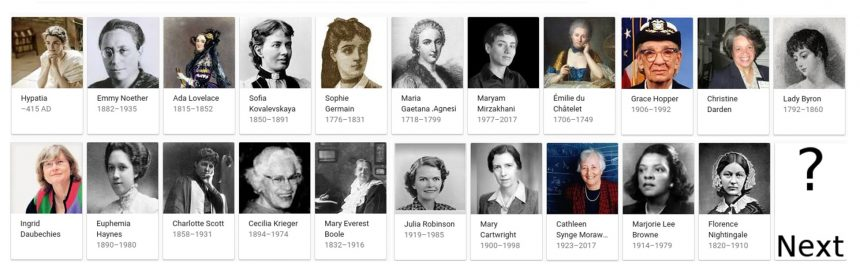I have to admit a reluctance to putting the modifier “Women” in this post, because it would seem to imply that on an absolute scale the mathematicians I mention here are not intrinsically great. Perhaps a better title would be The Greatest Mathematicians (who happen to be Women). In any case, it has always bothered me when I see girls and women either discouraged from or outright forbidden from becoming mathematicians. One way or another, it is I think a sign of our times that “mathematicians you’ve never heard of” is kind of redundant. I present these mathematicians in no particular order, for many reasons. Among those reasons is my personal opinion that the field of mathematics itself is not (again popular notions notwithstanding) like a vertical ladder, where first you learn counting, arithmetic, then algebra, geometry, trig, calculus and so on. In fact Mathematics, like Art, is more of a tree, with many branches, and many ways of thinking and seeing things. Some math is visual, some verbal, even some tactile. The fields these women pursued were likewise in many different areas, and their peculiar genius or accomplishment in each was profound. I won’t talk about all of the women pictured above, just the ones about which I would like to make a point. If you like, google “Greatest Women Mathematicians” for a very long and interesting list. ### Maryam Mirzakhani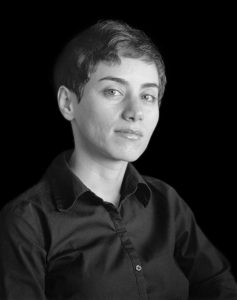When I was writing this piece this morning I was shocked and saddened to see that had just died last year (2017) of breast cancer. She was only 40, but had already done some profound work in geometry, especially Riemannian geometry — used by physicists in general relativity and elsewhere. She won the Fields Medal for her work in 2014, and became the first woman in history to win this award, described as the “Nobel Prize in Mathematics”. Maryam was born in Iran, and upon news of her death, a number of Iranian newspapers broke the taboo of printing a picture of her (a woman) with her hair uncovered. ### Cathleen Synge Morawetz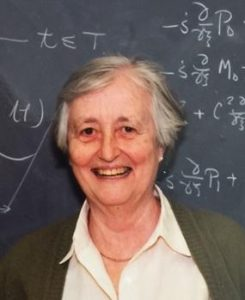Just one month after Maryam Mirzakhani died, we also lost Cathleen Morawetz (1923-2017), Professor Emeriti at New York University. Unlike most of the other mathematicians in this list, I had the great fortune to meet and get to know Cathleen in the 1980’s, while doing postdoc work at the Courant Institute in New York, where she at the time was the Director. I had gone to Courant to continue my studies of nonlinear wave equations, and Cathleen had made much of her own fame in that area, studying compressible fluids and shock waves. She was also the creator of the “Morawetz Inequality(ies)”, which have proven to have many uses, even to the understanding the stability of Black Holes. Cathleen was a very smart and jovial woman, and I will miss her. ### Emmy Noether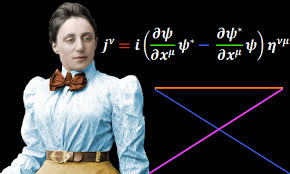Going back a bit, it would be difficult to convey just how profound and far-reaching was the work done by Emmy Noether, who lived from 1882 to 1935, and whose work touched many different branches of the tree of mathematics, including abstract algebra, geometry, and dynamical systems. One of the most profound theorems she proved (actually two with her name) is now known as Noether’s Theorem. What Noether’s (first) Theorem says is that for any conservation Law (such as energy, momentum, charge, etc), there is a fundamental geometric symmetry in the universe that corresponds to it. To express this poetically, Emmy proved that in mathematical physics, Truth (Law) is Beauty (Symmetry). Emmy’s Theorem resolved questions that Einstein had not been able to solve (!), and Einstein lobbied with Göttingen University (where she worked without pay or title) to promote her to a professorship. Eventually she was made professor, but with the rise of Nazi Germany soon had to leave the country for the US, due to her Jewish ancestry. ### Sofie Kovalevskaya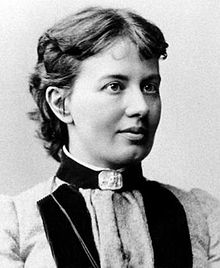Sofia “Sofie” Kovalevskaya lived from 1850 to 1891, and like Emmy Noether made substantial contributions to mathematical physics. She was a true pioneer, the first European female to earn a PhD in modern times. Together with Augustin Cauchy, she proved the Cauchy-Kovalevskaya Theorem, regarding the solutions to many equations in physics, especially those governing waves (light waves, sound waves, matter waves etc). Without her work I likely would not have had a job. Sofie was good in math but unlucky in love, her heart often broken. She had married and had children early on, and occasional star-crossed relationships later, but was also an early radical feminist and maintained a close and possibly romantic relationship with playwright Anne Charlotte Edgren-Leffler, the sister of Gosta Mittag-Leffler. Besides her main theorem, she was also the discoverer of what is now called the Kovalevskaya Top, an exact solution to a spinning top that completed work begun long ago by Euler and Lagrange. She was also a writer, and wrote “Nihilist Girl”, a semi-autobiographical work. “It is impossible to be a mathematician without being a poet in soul.” –Sofie Kovalevskaya. ### Florence Nightingale (Yes that Florence Nightingale) Besides being the founder of modern Nursing, Florence Nightingale had a knack for mathematics and especially statistics, and made great contributions in the visual display of quantitative information, a field which later was made popular by Edward Tufte in his seminal works. Ms. Nightingale was one of the first to make use of the Pie Chart, making clear causes and relationships in mortality among WWI soldiers. ### Hypatia There are so many others, such as Maria Gaetana Agnesi (the first woman appointed as full professor, but who died and like Mozart was buried in a pauper’s grave) but on my short list I have saved Hypatia for last. No likeness has ever been found, but she was said to be as beautiful as she was smart. The first documented female mathematician, Hypatia lived in 400 AD in Alexandria, and is considered by many to be the patron saint of mathematics. And a martyr. She was the daughter of the mathematician Theon, and inherited from him the position of Director of the Library of Alexandria, the ancient repository of world knowledge. Though Theon was considered a great geometer and wrote many treatises on Euclid, Hypatia was said to have surpassed her father in mathematics and astronomy, made astrolabes, and wrote many other works and commentaries on geometry. None of Hypatia’s works have survived, nor much of Library, whose destruction was considered one of the great tragedies in intellectual history. Hypatia was brutally assassinated by christian extremists, opposed to the “practice of sorcery, witchcraft, and mathematics”. Ironically, she was also a great teacher, and one of her most devoted students was Synesius, who studied under Hypatia as a neoplatonist, but eventually he converted to christianity and became a bishop, and contributed to the understanding of the doctrine of the Trinity. Hypatia fought hard to save the Library, but the world was changing and she could not stop it. So much was lost when the Library fell. With it was lost much knowledge, and science, and wisdom, that we will never recover. The fall of the Library presaged the Dark Ages. Had the Library stood, some have said, we might have landed on the moon in 1492, not just Florida. To be a woman. To be a scientist. To be a mathematician. All these things require more of one than any of us could ever know. Be brave, these women tell us. Be brave. ## You Will Be RememberedOn my Sunday training runs for the Zion Half Marathon, I usually go for a run up Utah SR 9, heading towards Zion from the town of Virgin. A few miles up, I pass by this gravestone just off the highway, the only remaining thing of the ghost town called Duncan’s retreat. I have never seen anybody stop here. Most people are tourists going 70 along this lonely stretch of highway, hell bent for Springdale lodging, and if they were looking anywhere it would be the other way, towards the Virgin River. A rock quarry lies just behind it, and nothing that would draw your attention to it. The history book has this to say about the long-gone town: A man named Chapman Duncan settled here in 1861. Shortly after several other families moved here also. In 1862 the Virgin river flooded and destroyed most the town. A lot of the people moved away but new settlers came. By January of 1863 about 70 people lived here. In 1863 a post office was built, a school was built in 1864 along with a meeting house. In 1866 floods took its toll on this town also and over the next few years high water from the Virgin river destroyed the fields and killed the town. By 1891 the town was deserted. All that remains of the town today is a grave of a lady named Nancy Ferguson Ott who died here in town in 1863. Her grave is located on the north side of highway 9. (Submitted by Bob Bezzant.) My understanding from other locals is that Duncan used to live in our town of Virgin (now population 600), but “retreated” to this place far from Virgin to get away from the bustling metropolis. When Nancy Ferguson Ott died, Duncan’s Retreat had 70 citizens, a post office, families, friends. It was a town, and they thought it would stick around long enough for there to be a cemetery with more than just Nancy, but Nature and circumstance had other ideas. Now she is utterly alone, on a lonely stretch of anonymous highway, with no one around, no town, no post office, no friends no family. I stopped to look at the grave marker. There are fresh flowers, stuffed animals, cards. They are replaced regularly by somebody somewhere. I do not know who. I take some comfort in this, and I hope you do too. No matter who you are, or how alone you may feel in this world, Nancy Ferguson Ott is here, on State Route 9, miles from anywhere and anyone, to deliver this message to you: No one dies alone. You will be Loved, You will be Remembered. ## Relativity, Simplified. Really.I’ll make this (mercifully) short. Consider this statement, which I call Ω:  Everything travels through the Cosmos at exactly the speed of light. Ω is Relativity. That’s it. Really, it is. And Ω is true not only for Special Relativity, but the General one too. All you have to know is that the “Cosmos” means both space and time. If you were to ask somebody what Relativity says, they would probably say something like “everything is relative.” The problem with that is, it’s not precise. In fact, it’s not even true. Some things in physics (and the world) are thought to be absolute. So I’ve been trying to come up with a good version of Relativity that can be justified mathematically, and that little box is what I came up with. If you understand what each word means exactly, everything follows from this statement, and if you like you can stop reading now and get on with life. Because that really is what relativity says. The rest of this short post is just me rambling about why I like it. In a later post I’ll defend it. Also, here is a cool picture of a galaxy for no reason. But it’s cool (*). ### Why I like This Version of Relativity One of the things I like about this version (Ω) is that it is simple and precise, sounds a little strange — but just enough strange to be right — and answers immediately a number of other questions people ask about relativity, matter, speeds etc. For example: Q: Will we ever be able to go faster than light? A: NO. The reason you can’t go faster than the speed of light is that you can’t go any slower either. Nothing in the universe can go any speed in spacetime but c, the speed of light. The only thing you can ever change is the direction in space-time that you are going. Q: Why does Relativity say that when you go faster, time slows down? A: Because you are always going exactly at the speed c, so if you go faster in the space directions, you have to go slower in the time direction so it still adds up to exactly c. See? Details later, film (and math) at 11. But really, it is true. Ask a physicist. He’ll scratch his head, then say yeah, that works, then go have a beer. (*) Photo: (Image Credit: X-ray: NASA/CXC/CfA/R.Kraft et al.; Submillimeter: MPIfR/ESO/APEX/A.Weiss et al.; Optical: ESO/WFI) ## An Anti-Christmas Carol Tomorrow will be June 25, the orbital opposite of December 25, and making us nearly as far as possible astronomically from the spirit of Christmas as we can be. Seems appropriate then, with the current sentiment pervading our leadership these days regarding the poor, the young, the sick and the elderly, and the changes proposed to the way our society treats these people. It therefore seems appropriate to publish here an excerpt from Dicken’s classic story, reflecting what seems to be the mood of the times, which is to tell Tiny Tim that he is a freeloader, and needs to pull himself up by his bootstraps and get a job. Or just go away and die. Merry Christmas, Everyone. ## A Christmas Carol – Marley’s Ghost “At this festive season of the year, Mr. Scrooge,” said the gentleman, taking up a pen, “it is more than usually desirable that we should make some slight provision for the Poor and destitute, who suffer greatly at the present time. Many thousands are in want of common necessaries; hundreds of thousands are in want of common comforts, sir.” “Are there no prisons?” asked Scrooge. “Plenty of prisons,” said the gentleman, laying down the pen again. “And the Union workhouses?” demanded Scrooge. “Are they still in operation?” “They are. Still,” returned the gentleman, “I wish I could say they were not.” “The Treadmill and the Poor Law are in full vigour, then?” said Scrooge. “Both very busy, sir.” “Oh! I was afraid, from what you said at first, that something had occurred to stop them in their useful course,” said Scrooge. “I’m very glad to hear it.” “Under the impression that they scarcely furnish Christian cheer of mind or body to the multitude,” returned the gentleman, “a few of us are endeavouring to raise a fund to buy the Poor some meat and drink, and means of warmth. We choose this time, because it is a time, of all others, when Want is keenly felt, and Abundance rejoices. What shall I put you down for?” “Nothing!” Scrooge replied. “You wish to be anonymous?” “I wish to be left alone,” said Scrooge. “Since you ask me what I wish, gentlemen, that is my answer. I don’t make merry myself at Christmas and I can’t afford to make idle people merry. I help to support the establishments I have mentioned—they cost enough; and those who are badly off must go there.” “Many can’t go there; and many would rather die.” “If they would rather die,” said Scrooge, “they had better do it, and decrease the surplus population. Besides—excuse me—I don’t know that.” “But you might know it,” observed the gentleman. “It’s not my business,” Scrooge returned. “It’s enough for a man to understand his own business, and not to interfere with other people’s. Mine occupies me constantly. Good afternoon, gentlemen!” ## The Seven Billion Species of ManAs of the moment of this writing, there were currently 7,509,287,532 people alive on the planet. (source: World Odometer). Each of these people include themselves as members of various subgroups, such as Men, Women, Caucasian, Native American, Bipolar, Russian, Spectrum Autistic Syndrome, LGBTQ, Republican, Democratic, Episcopalian, Atheist, USC Alumni, Rotary Club, ISIS, Down’s Syndrome, and others. Each of these groups has their own collection of stories, which include origin stories (how they came to be), membership stories (who is qualified to be a member), enemy stories (with whom they have fights and why), and stories about why their group is so much better than all the others. All of these stories are important to the members of those groups. Some stories are so important to those members that they are willing to fight and die for that story. Even those whose stories are to anybody outside the group clearly arbitrary (such as Professional Football Team fans), people get into mortal combat over a bad referee call, with another human being whose only difference is the color of the shirt favored by that team over the other. Recently there have been many arguments (especially in academic circles) around the questions of ethnicity, sensitivity to other cultures, and offenses (real or imagined) taken by somebody when someone else “appropriates” the others culture, tribe, race, religion, or other group membership. This includes such things as “whitewashing” a dramatic or historical role (e.g. by a person associated with one “race” portraying an historical character of another “race”). These controversies become especially heated when one of the groups has historically been oppressed, attacked, targeted for genocide etc by another group in power. The tragedy of all these stories, both of the oppression, the suffering, and of the later push-back by the oppressed groups, is that they are all based on stories, as as stories, they are fiction, and as fiction, they are far from the actual reality on the ground, at least to the best that science can discern. Here is the story that evolutionary science suggests may be a more accurate story about the species we call Man:  Every single 'Human' born is a mutation, and may even be a brand new species. In other words, none of us are the same, and any allegiance to a particular group, religion, ethnicity or other collection of humans is arbitrary, and made up only of stories, nothing more. The earth is not populated with over seven billion humans. It is populated with over seven billion individuals, each a unique experiment of Nature, bearing only incidental similarities in physical, sexual, psychological or other features with others which whom they may share social or familial descendancy. This is the general perspective I have been gradually coming to embrace. Perhaps it is easier for me, because I have never particularly felt like I belonged to any group, race, or religion. Almost certainly it is also due to what others call “privilege,” in that because of my outward appearance of whiteness, the dominant ethnic group of society in America does not constantly harass or subdue me because of my differences. It is quite possible that I would tell a different story if my skin were a different shade. When under assault, groups of people unite out of self-defense, and have only their shared oppression to bind them together. And yet, regardless of that fact, the evidence remains that the only story that truly seems to be the case is one, not of races and ethnicities, but of radical individualism, that we are each a member of groups which have only one member in them, and that all other associations are pure fictions, what Kurt Vonnegut Jr. called “Granfalloons“, which are arbitrary and pointless associations that bind people together. To be aware of this absurd situation may seem depressing, but over the years, I have found it to be liberating, and ironically joins me in brotherhood with all other humans, with the great Granfalloon, based on the fact that we are all utterly alone. And now at the end of this piece, the population has risen to 7,509,296,070 people. I would like to welcome these new 8538 creatures to the earth, and wish them each well in their unique journey through life, and to remind them not to take the stories told to them too seriously. ## Automotive MathCalculus is taught all wrong and too late. The basics could be taught in the car on the way to Kindergarten. Here is how it goes. Any kid who’s been in a car knows that the speedometer tells you how fast you are going, and the numbers in the odometer (mileage) tells you how far you’ve gone. Another way to say that is that the speedometer shows how quickly the odometer is changing. And another way to say that is that the speedometer is the “differential” of the odometer. To be fancy, we can use a small “∂” for differential and so we say: $$Speedometer = ∂\,{Odometer}$$ That’s differential calculus. How things change. Subtraction. Put another way again, the odometer tells you what the Sum total distance was after going all those speeds that the speedometer indicated. Instead of saying “Sum” with a big S we stretch it out into a long skinny S like this:$\int\$ and we say

$$Odometer = \int { Speedometer }$$

That’s integral calculus. How changes accumulate. Addition.

Subtraction undoes addition. That is the Fundamental Theorem of Calculus. Duh. Next stop, Rocket Science !

THE END.

1 2 3 7